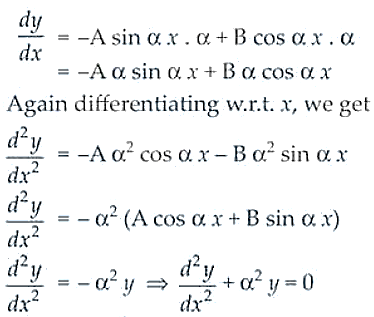# NCERT Exemplar Solutions for Class 12 Maths Chapter 9 Differential Equations

The problems of NCERT Exemplars are important for students, as they help them understand the different question variations and, thus, develop their problem-solving abilities. NCERT Exemplar Solutions, created by subject matter experts at BYJU’S, provide step-by-step solutions to the exercise problems of all chapters. All these solutions have been created, keeping in mind the latest CBSE guidelines, in order to help students score high marks.

Chapter 9 of NCERT Exemplar Solutions for Class 12 Maths is Differential Equations explains concepts of differential equations, such as degree and order of differential equations, to form a differential equation that will represent a given family of curves, how to solve First Order and First Degree by differential equations methods, differential equations with variables separable, homogeneous differential equations and linear differential equations. Students who need guidance in solving the exercise problems of this chapter can access the PDF of NCERT Exemplar Solutions for Class 12 Maths Chapter 9 Differential Equations from the link below.

## Download the PDF of NCERT Exemplar Solutions for Class 12 Maths Chapter 9 Differential Equations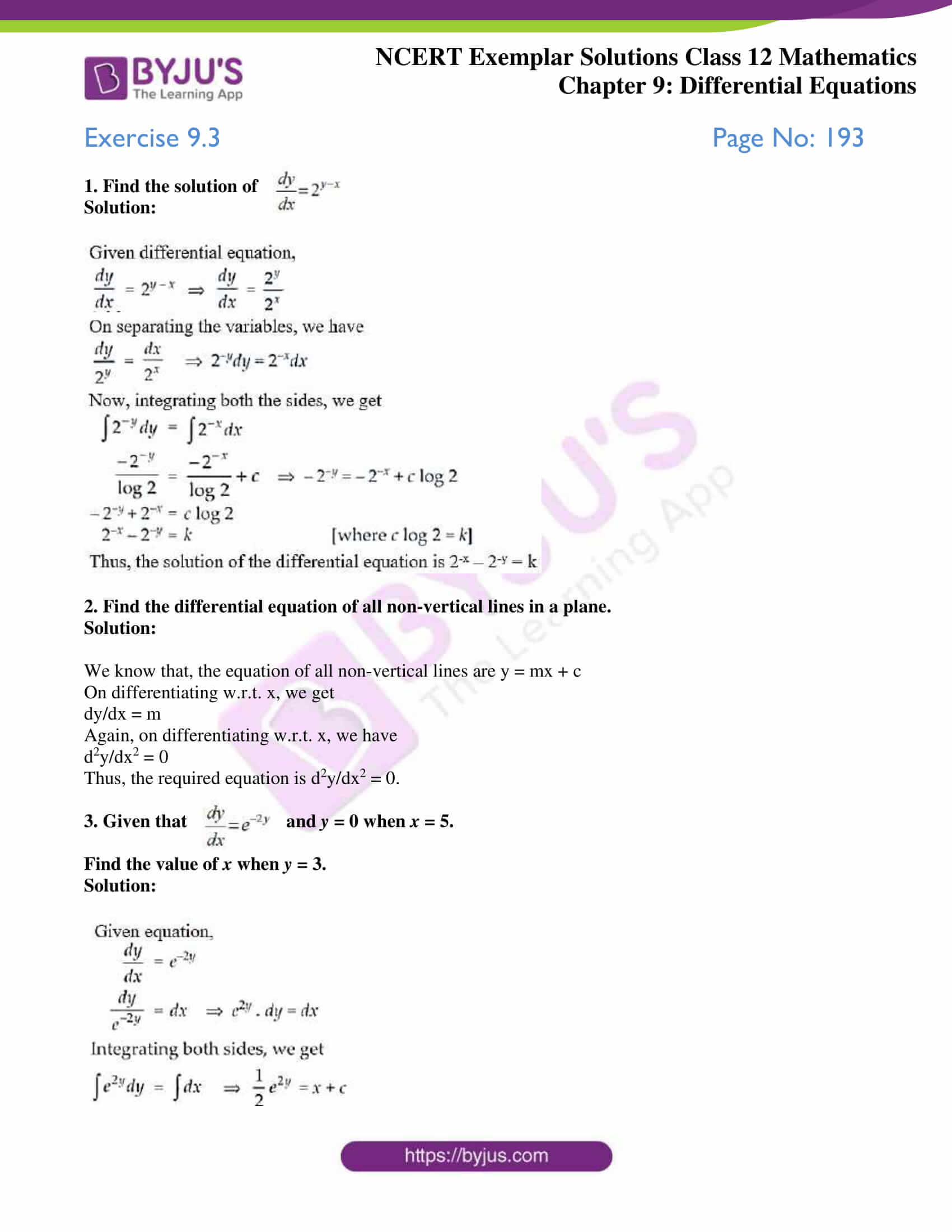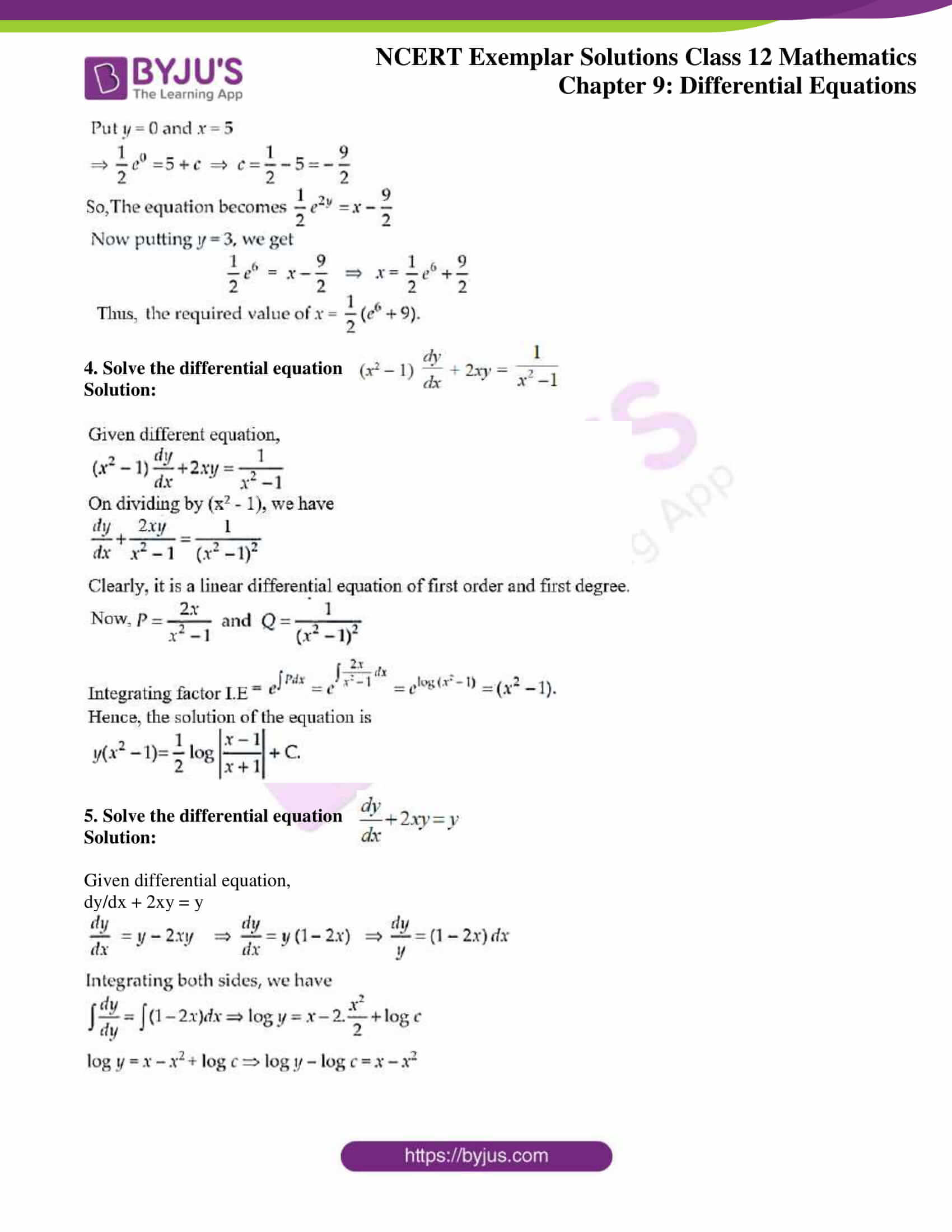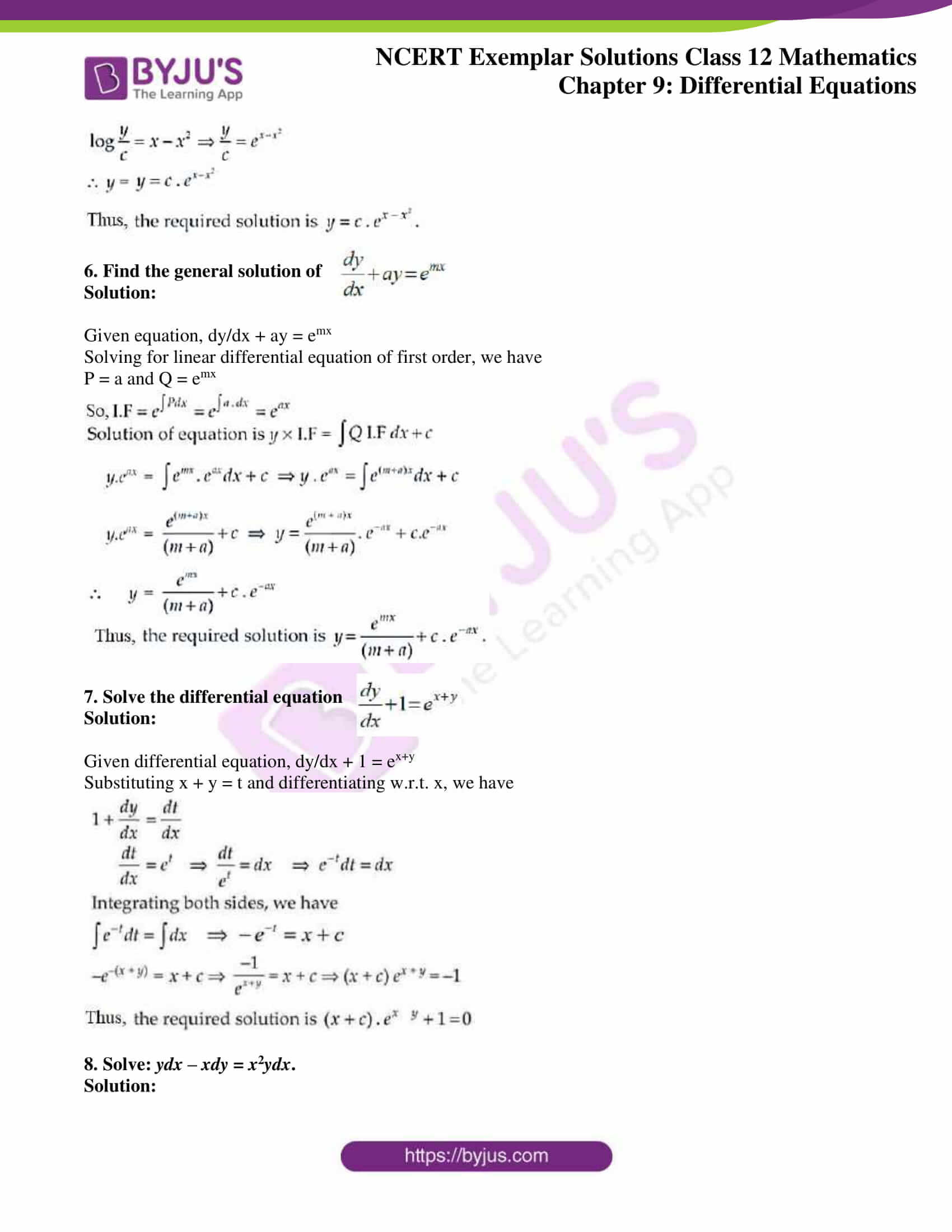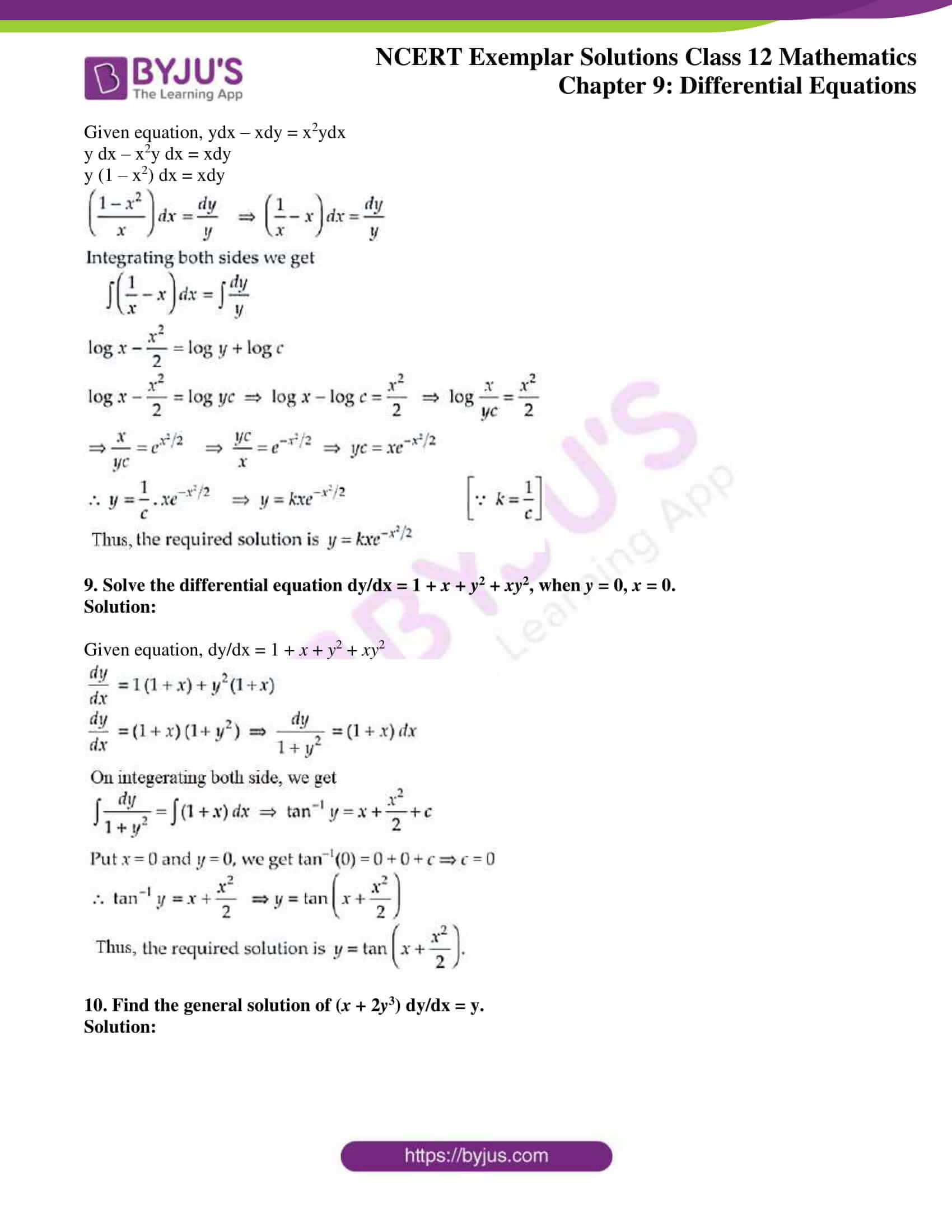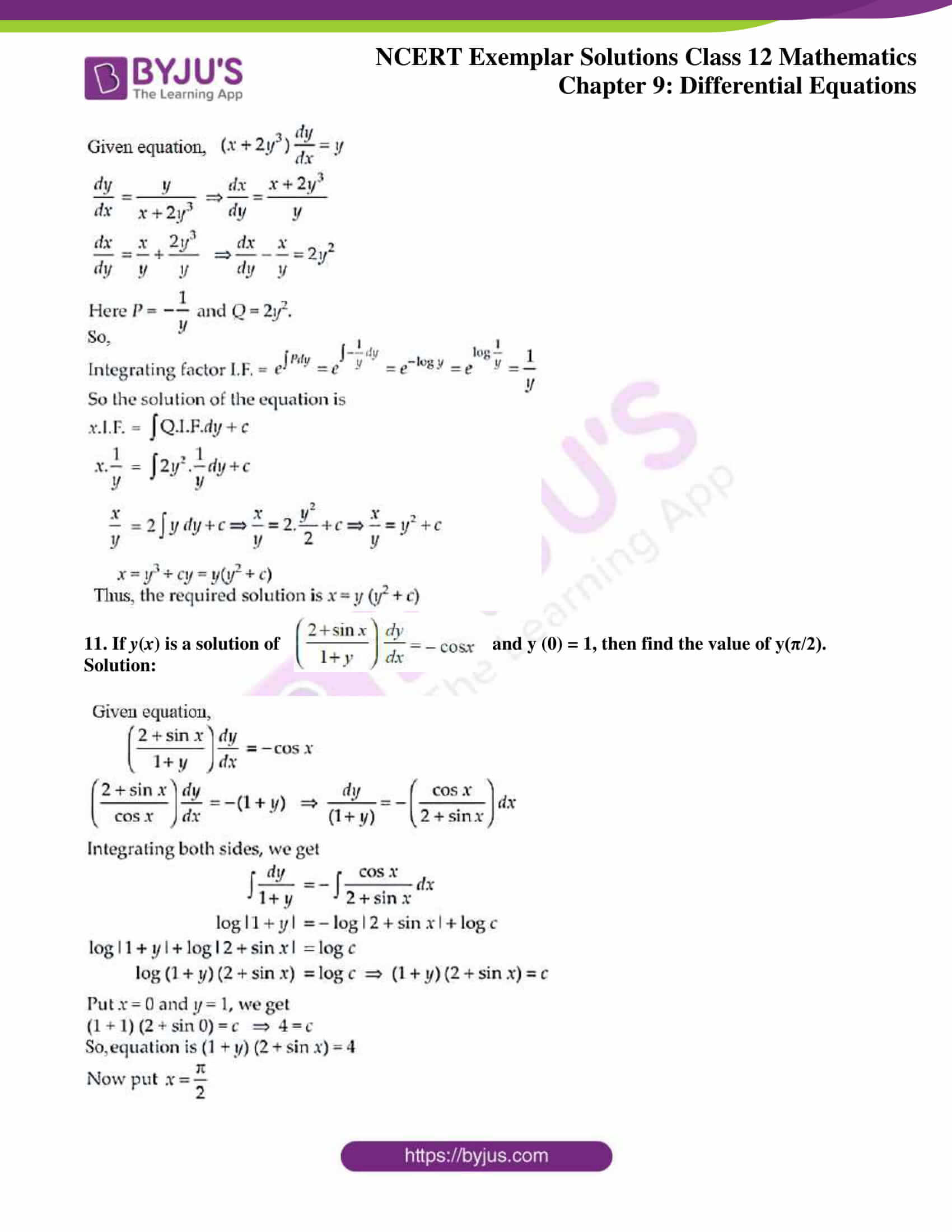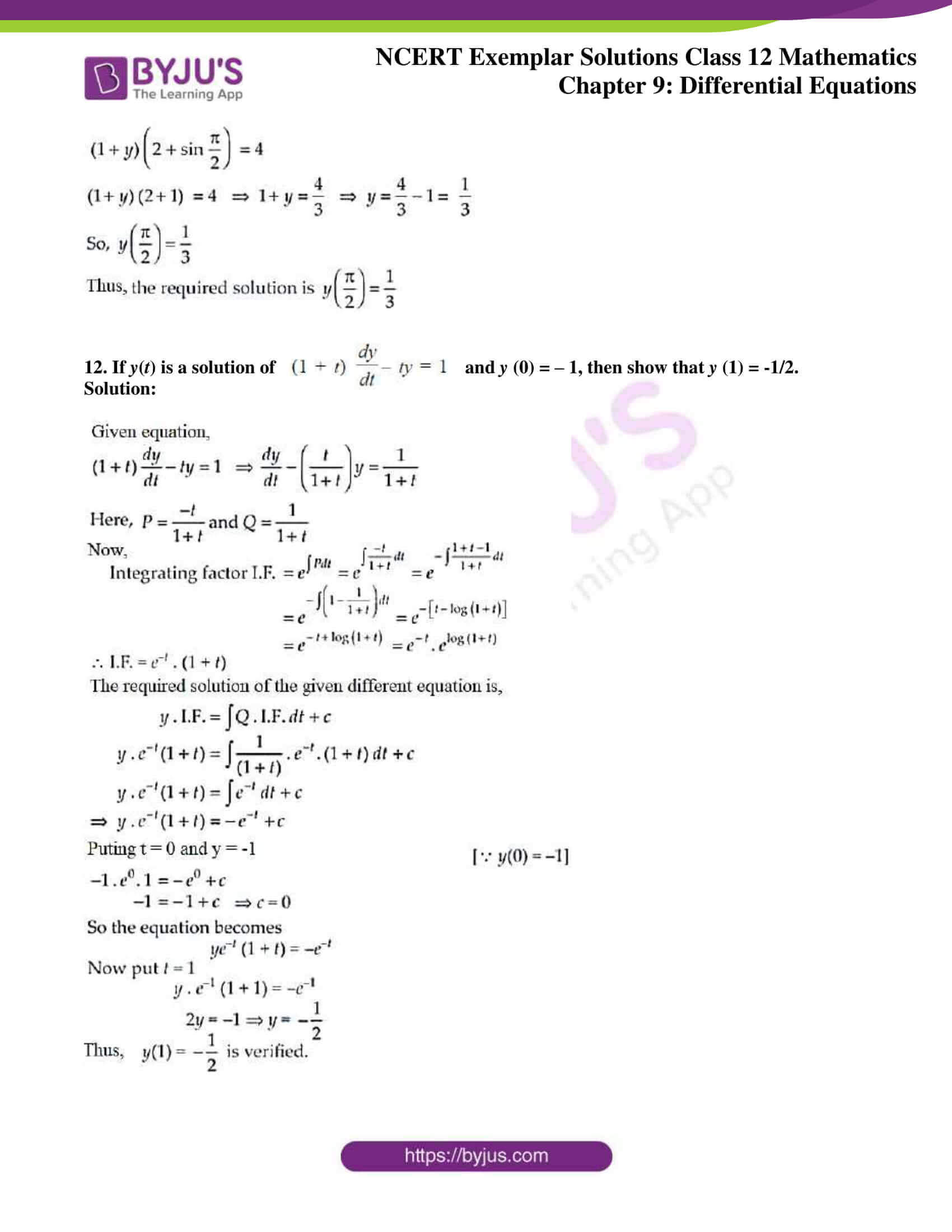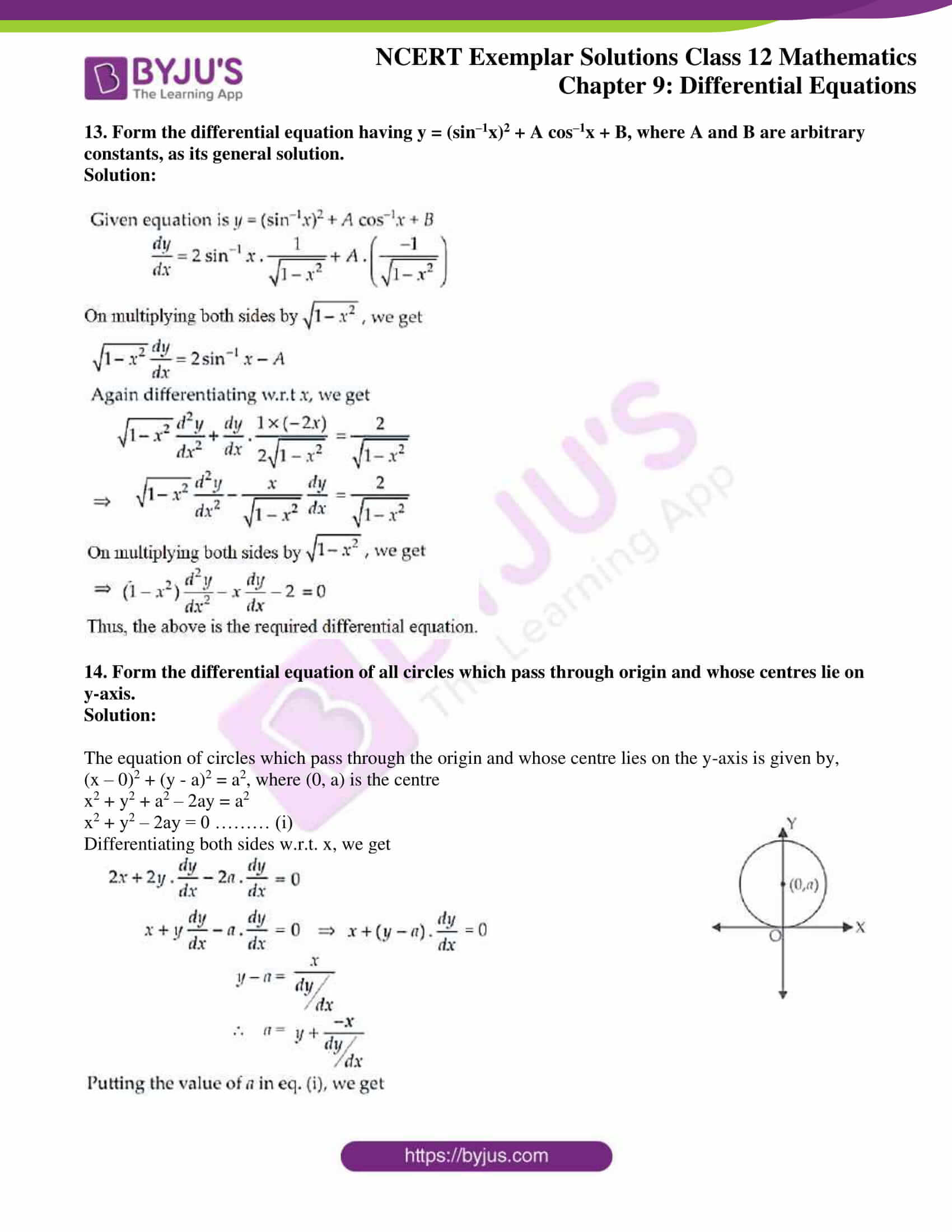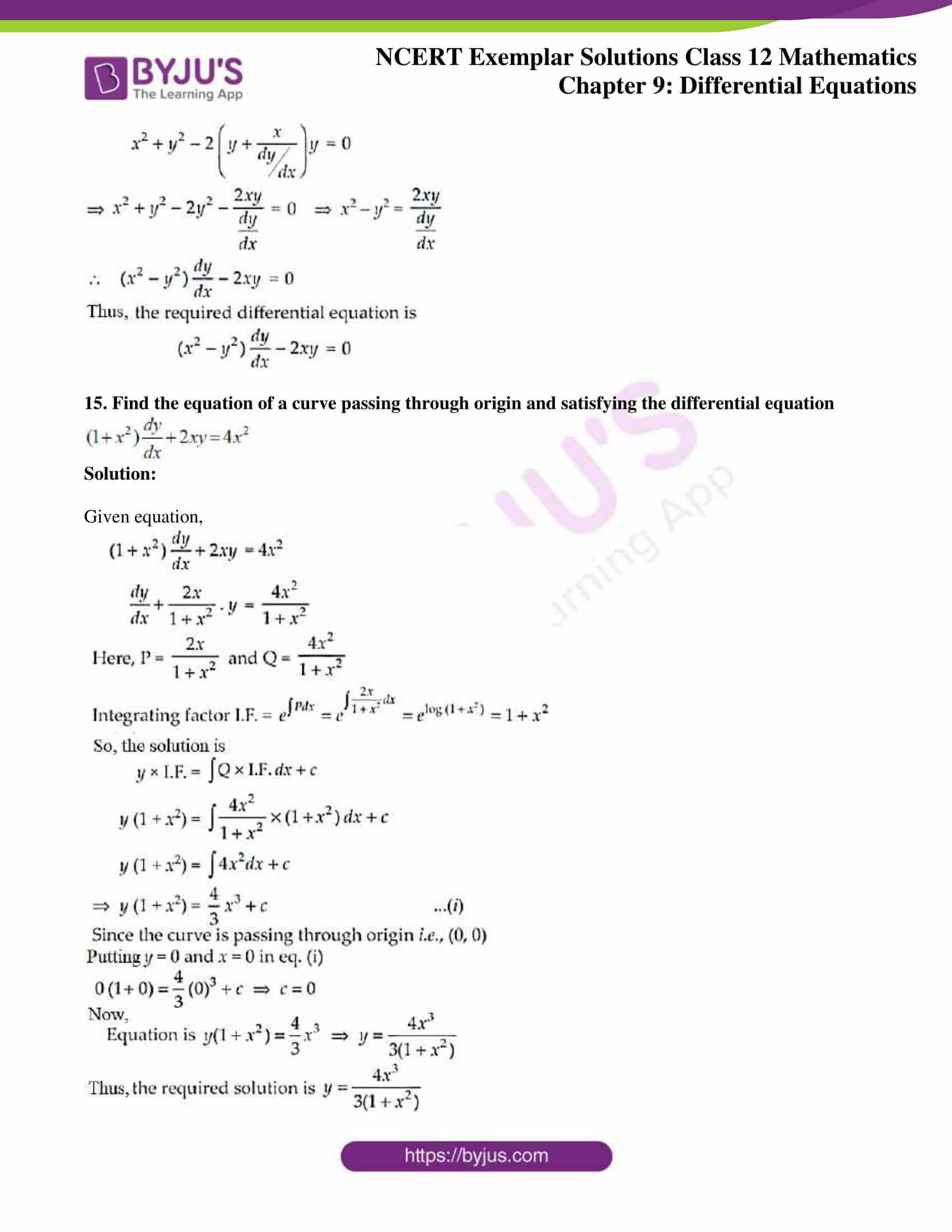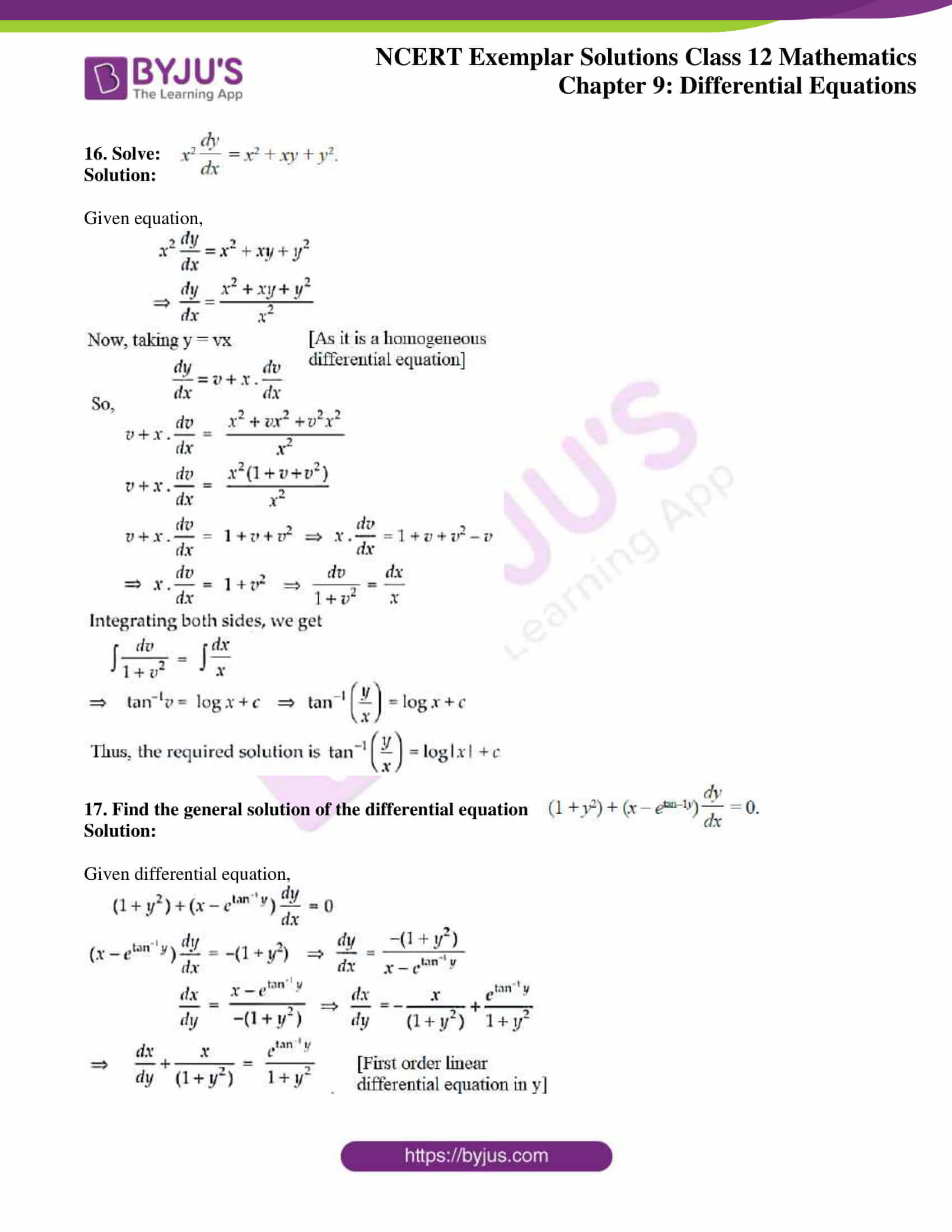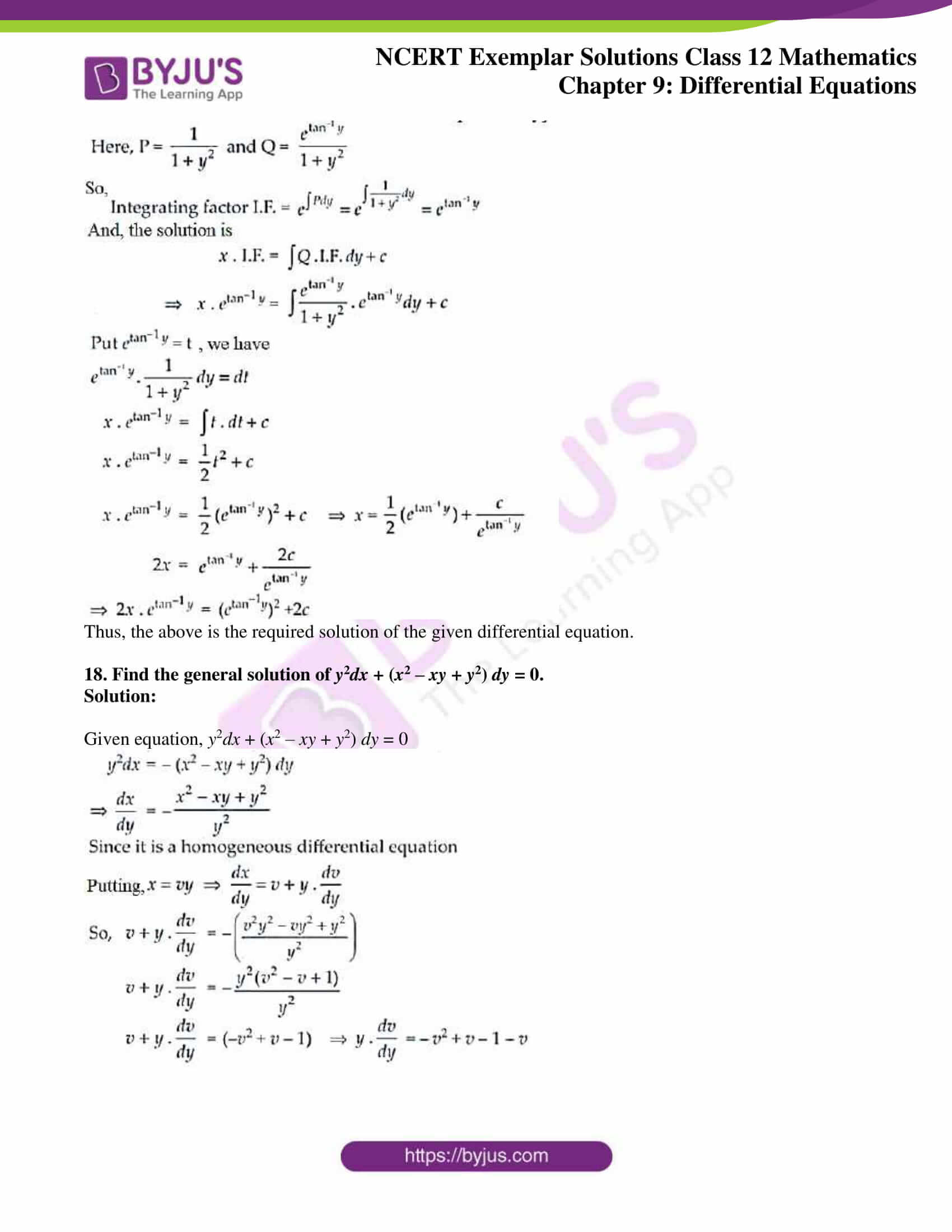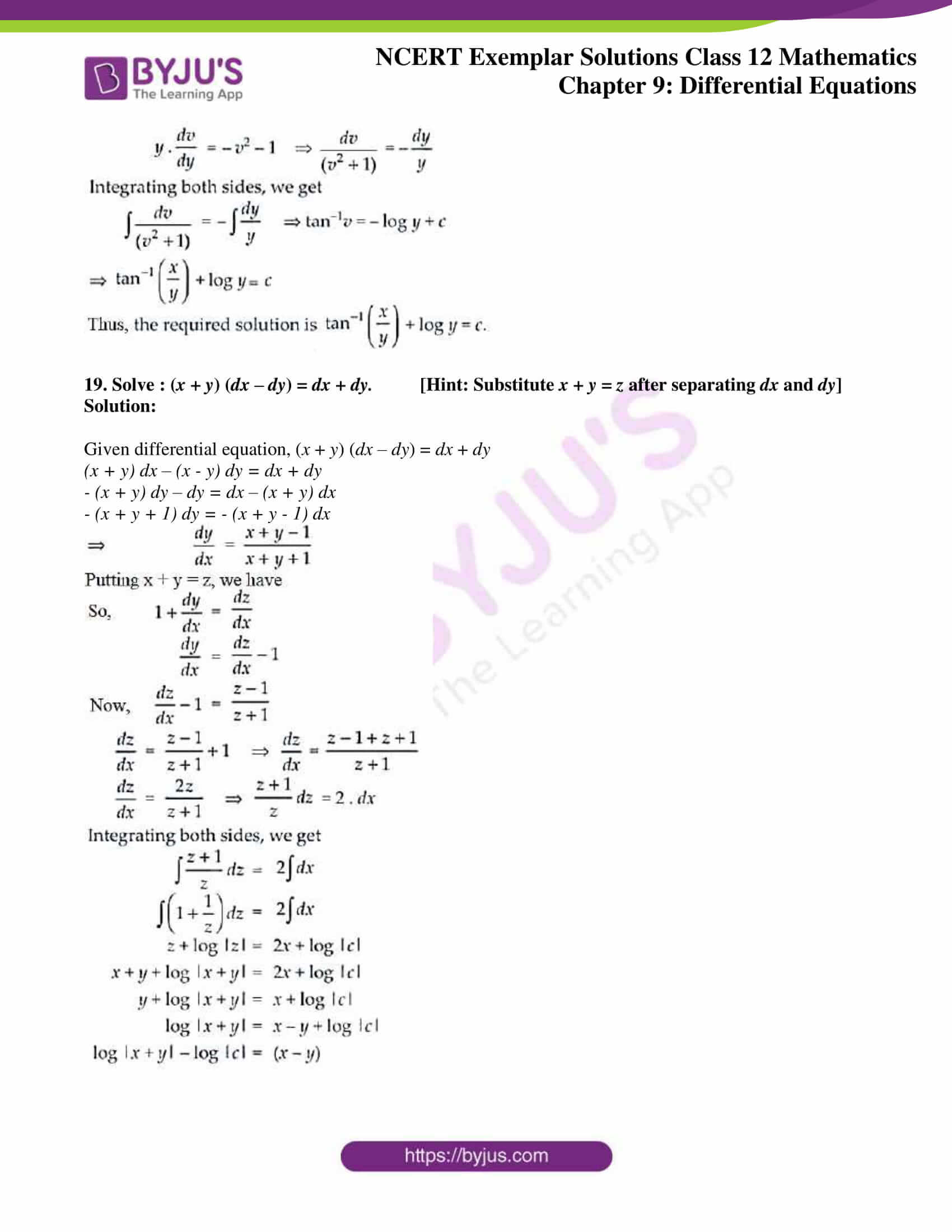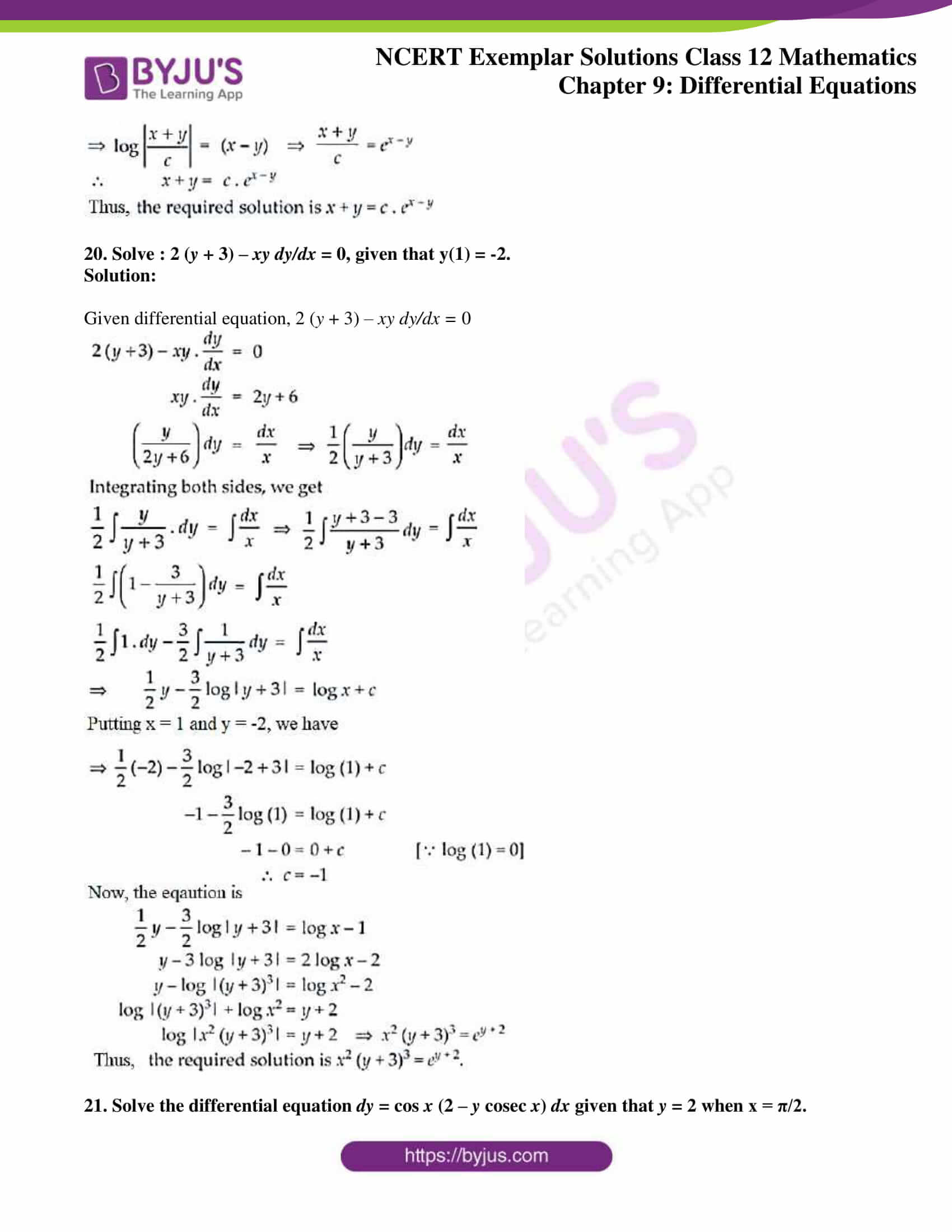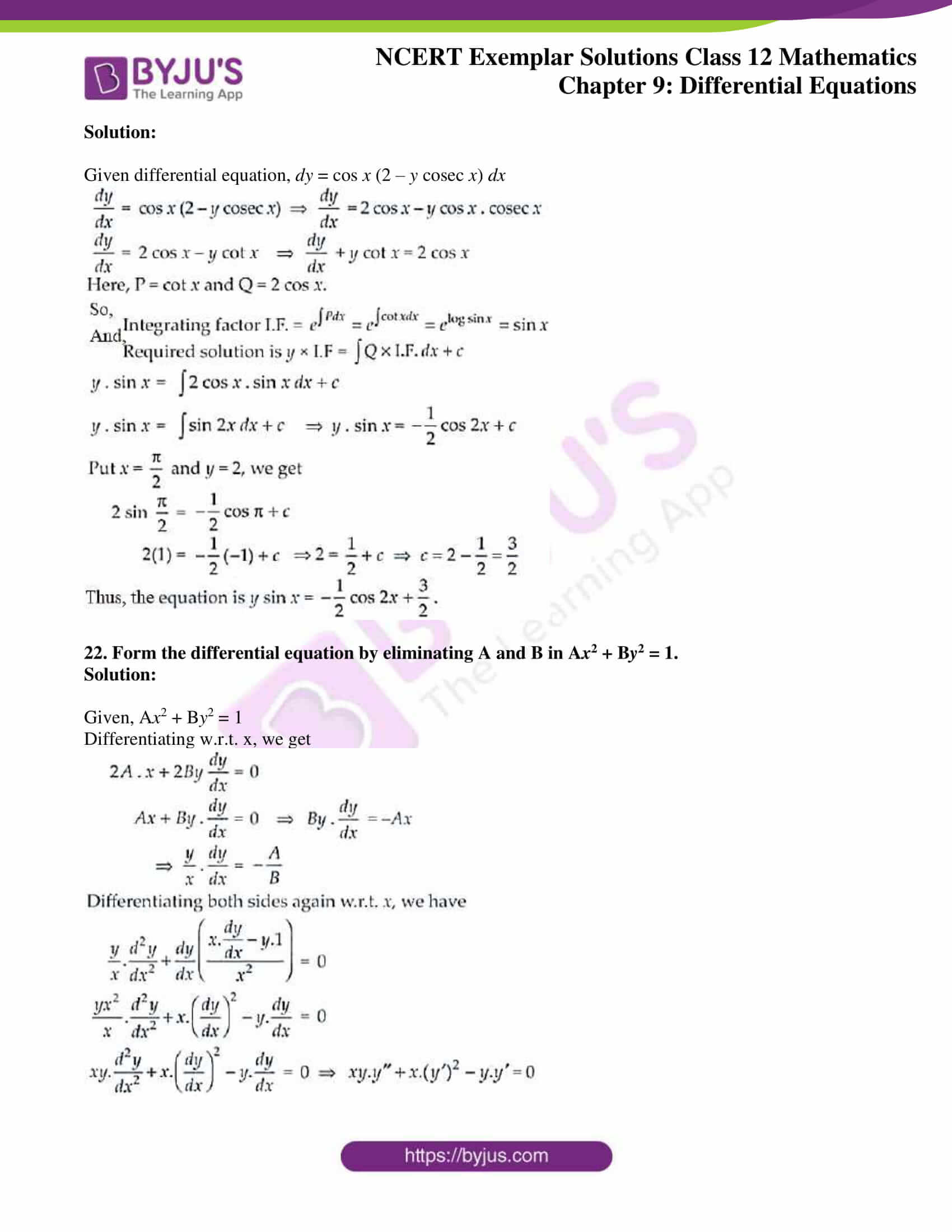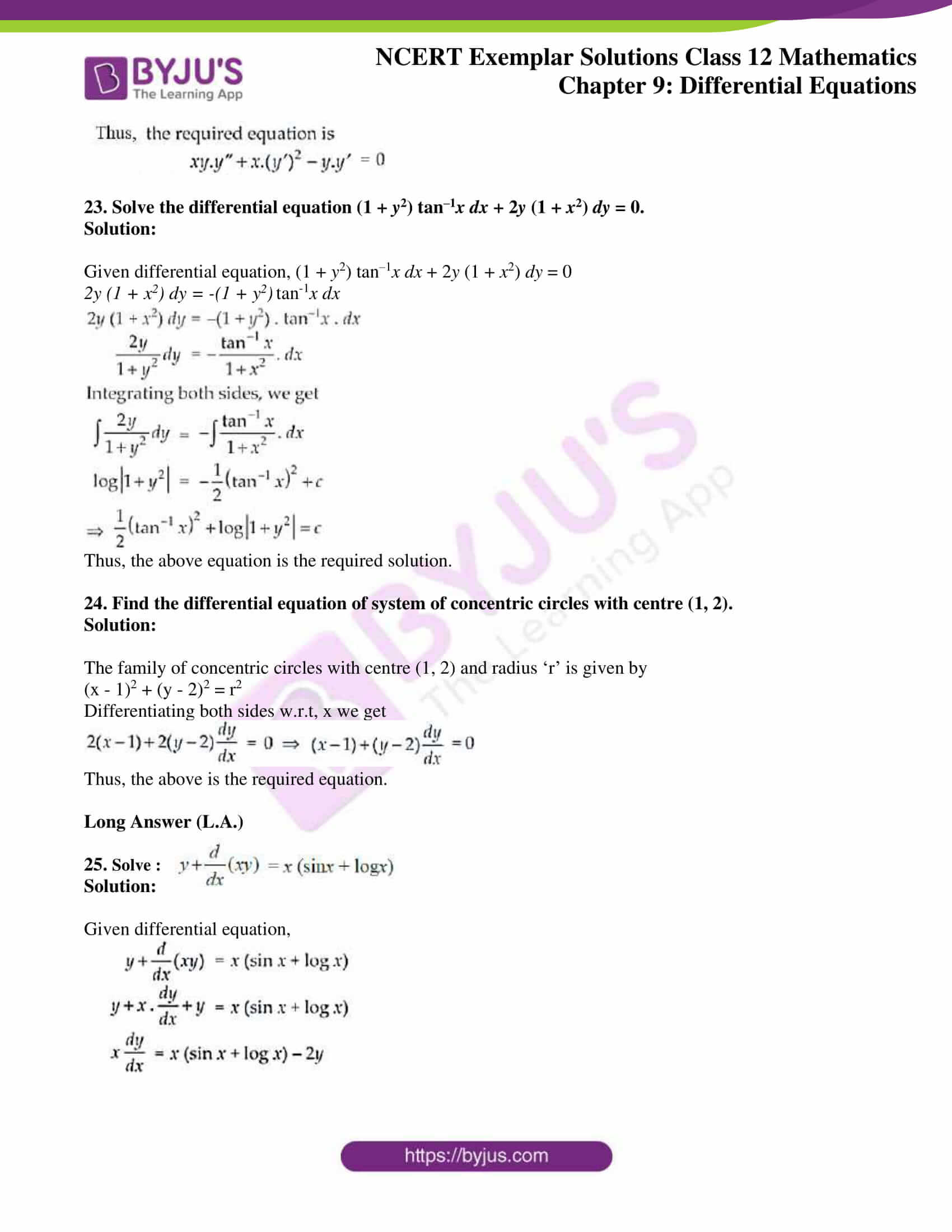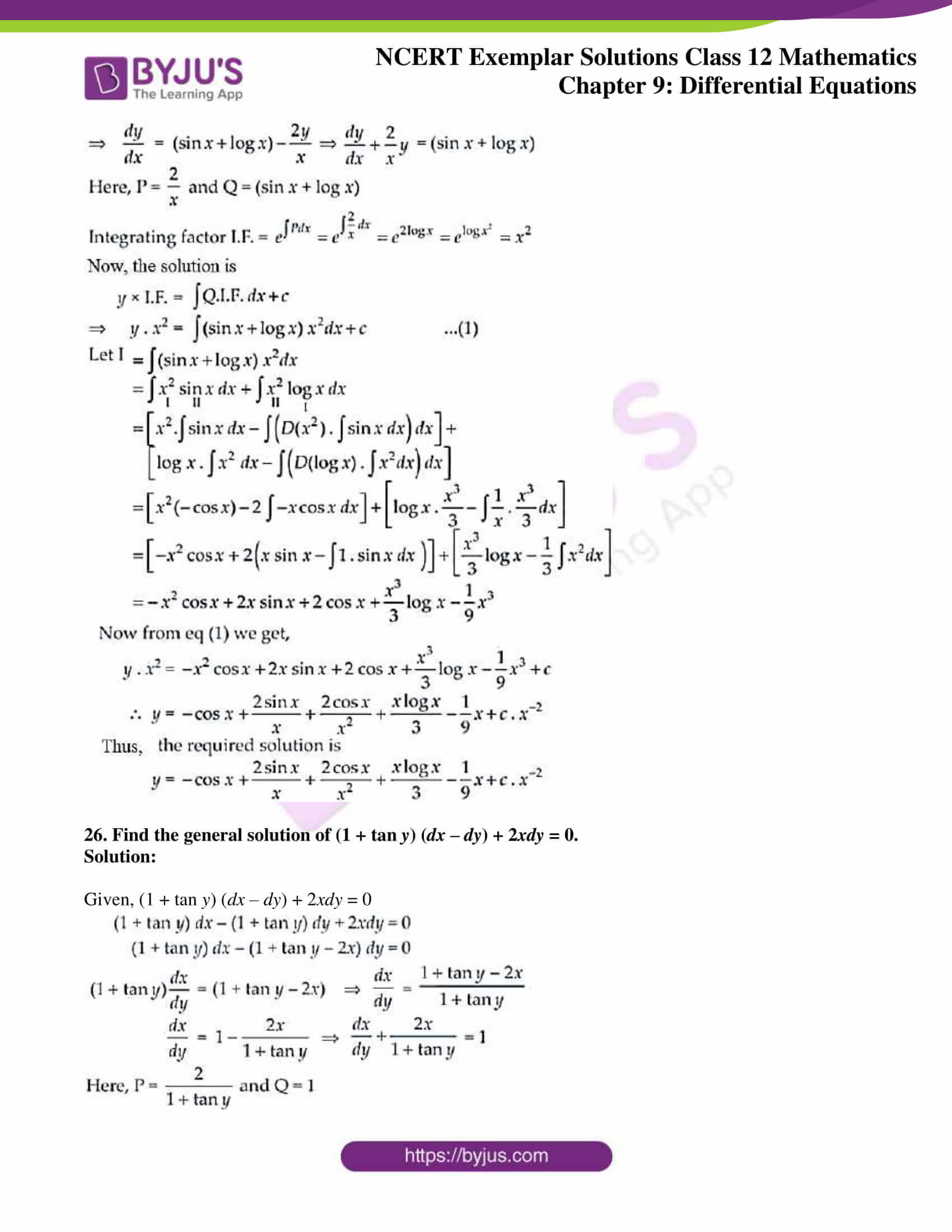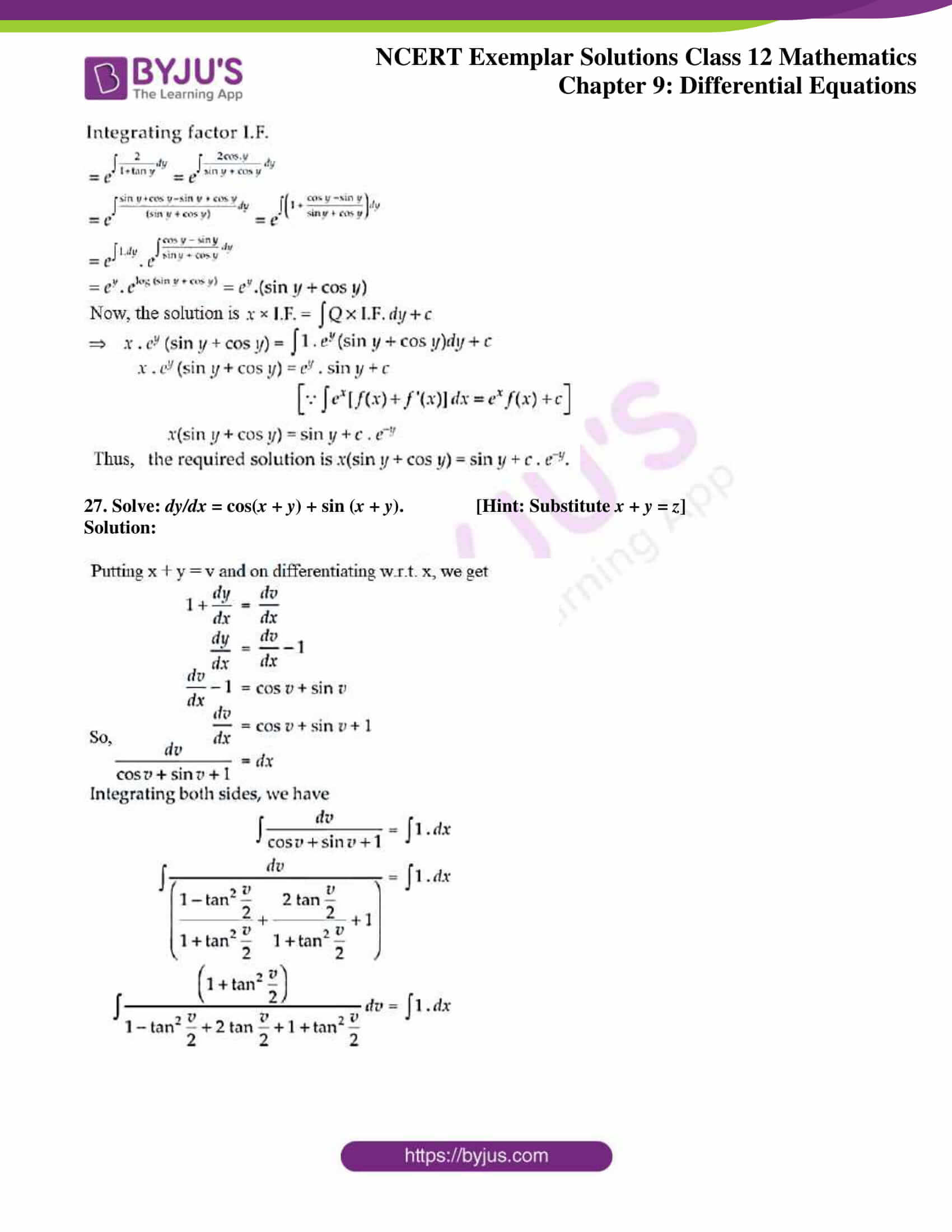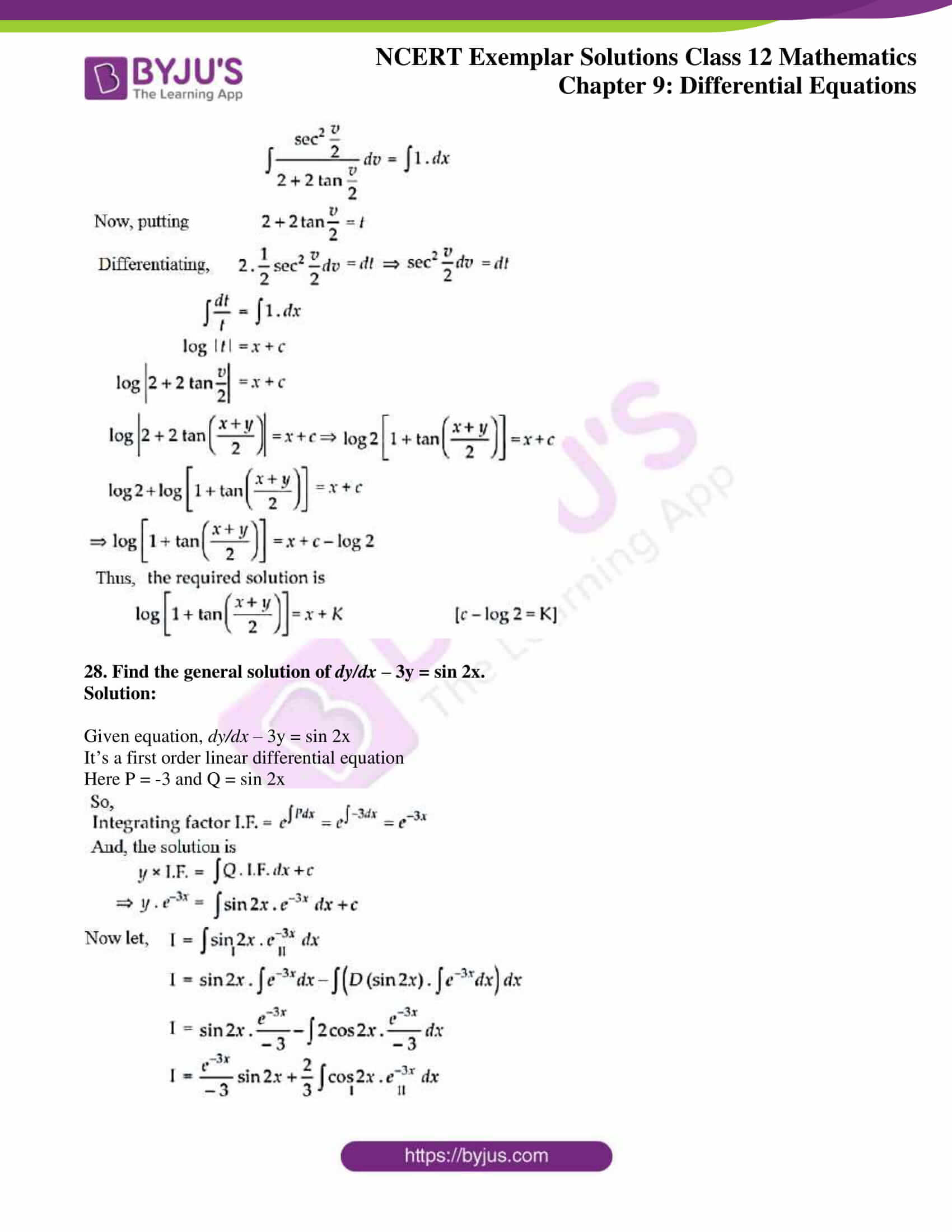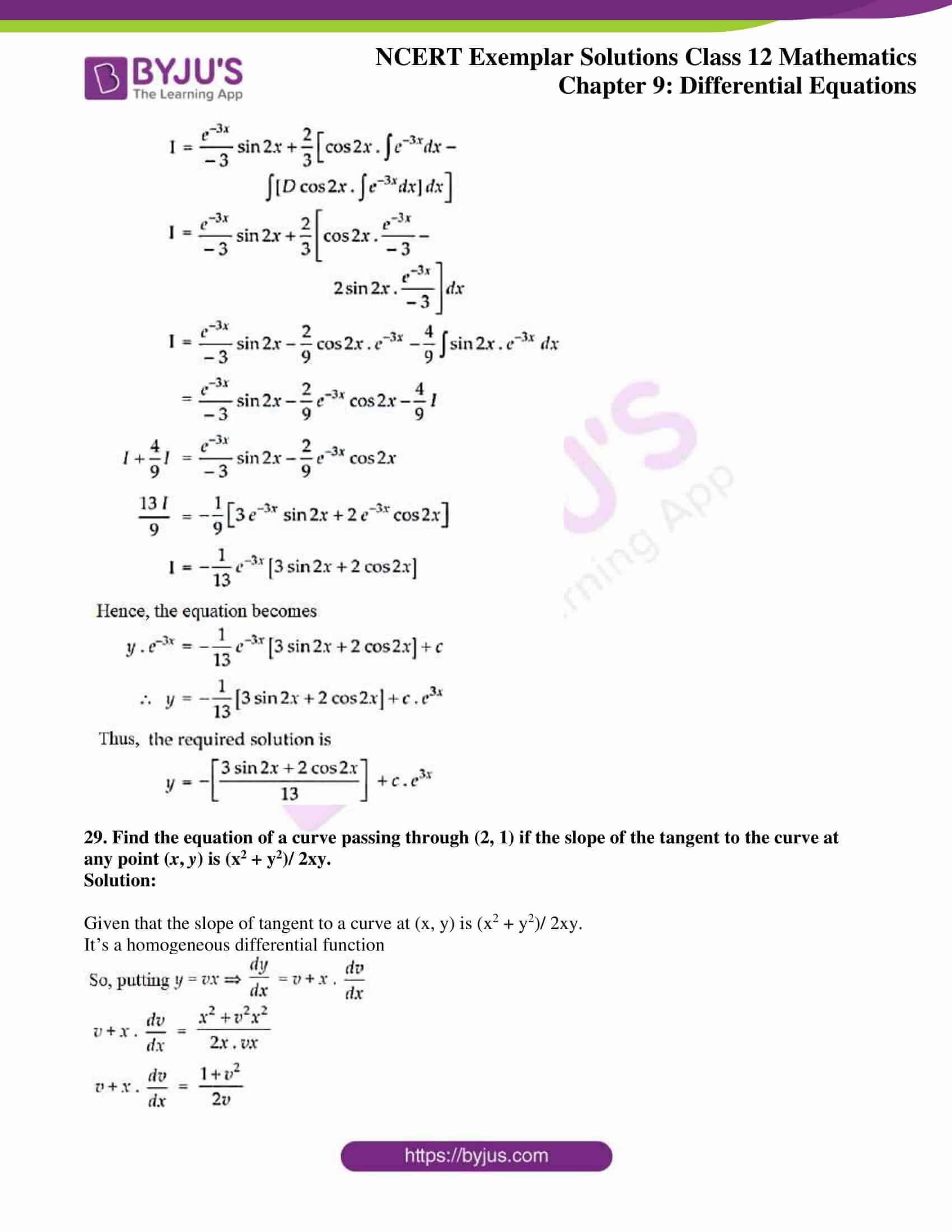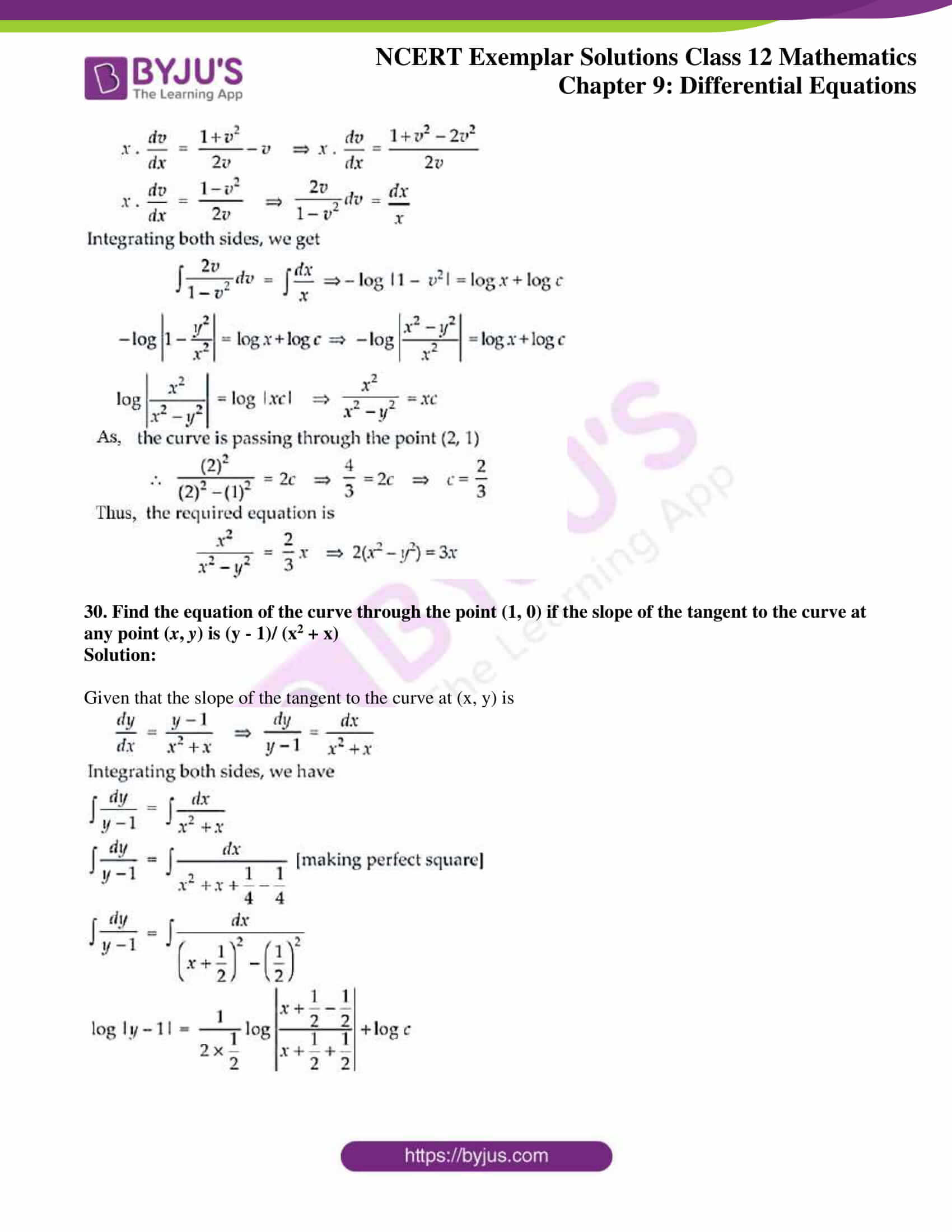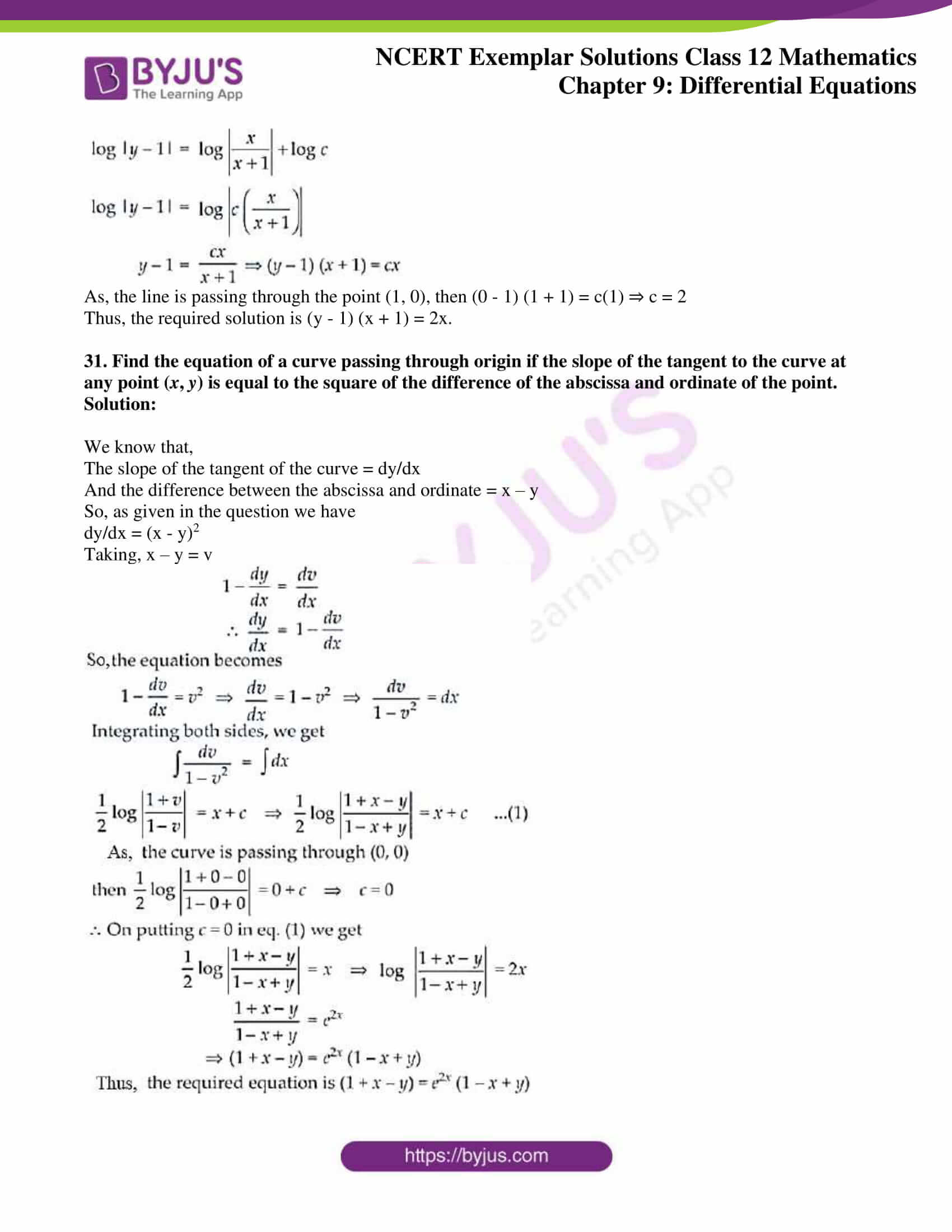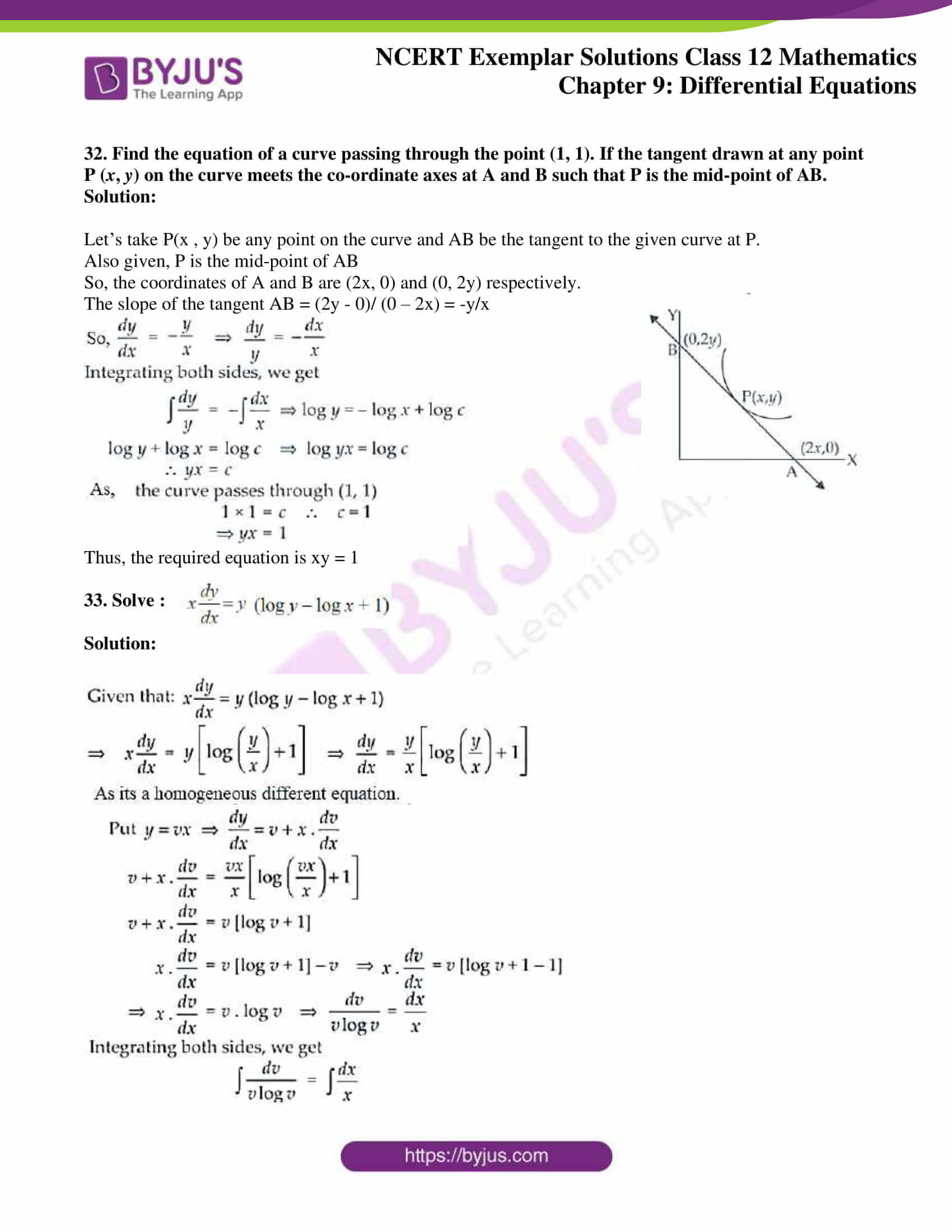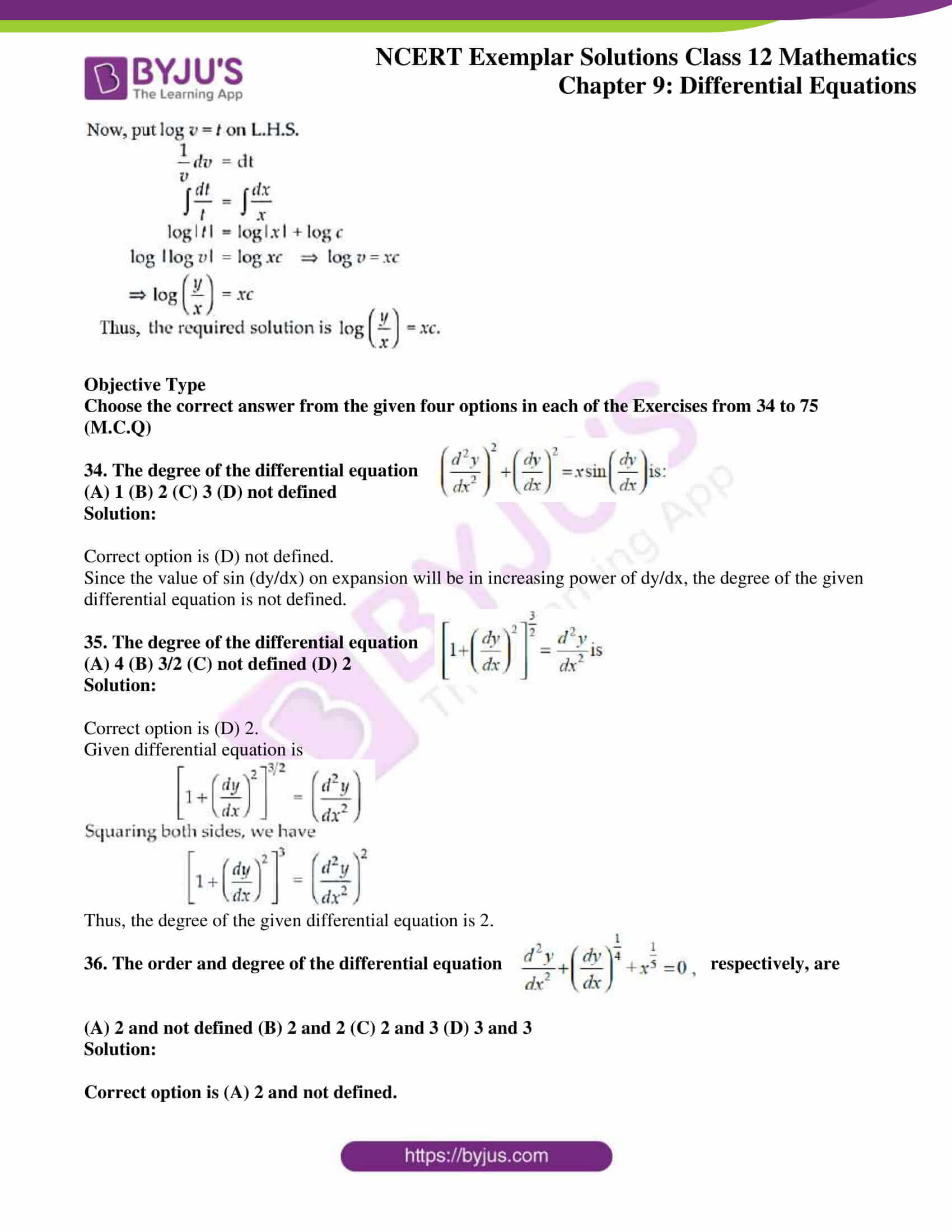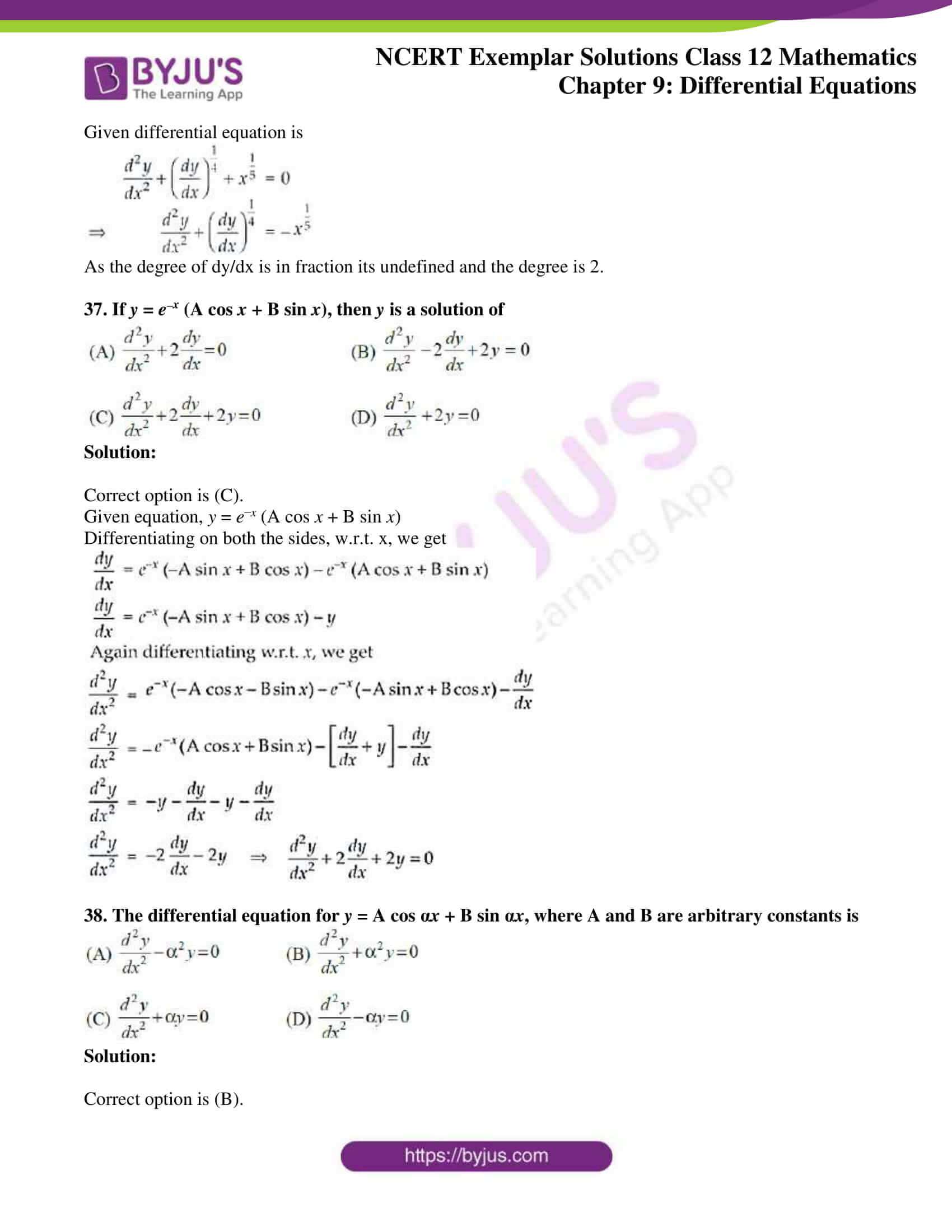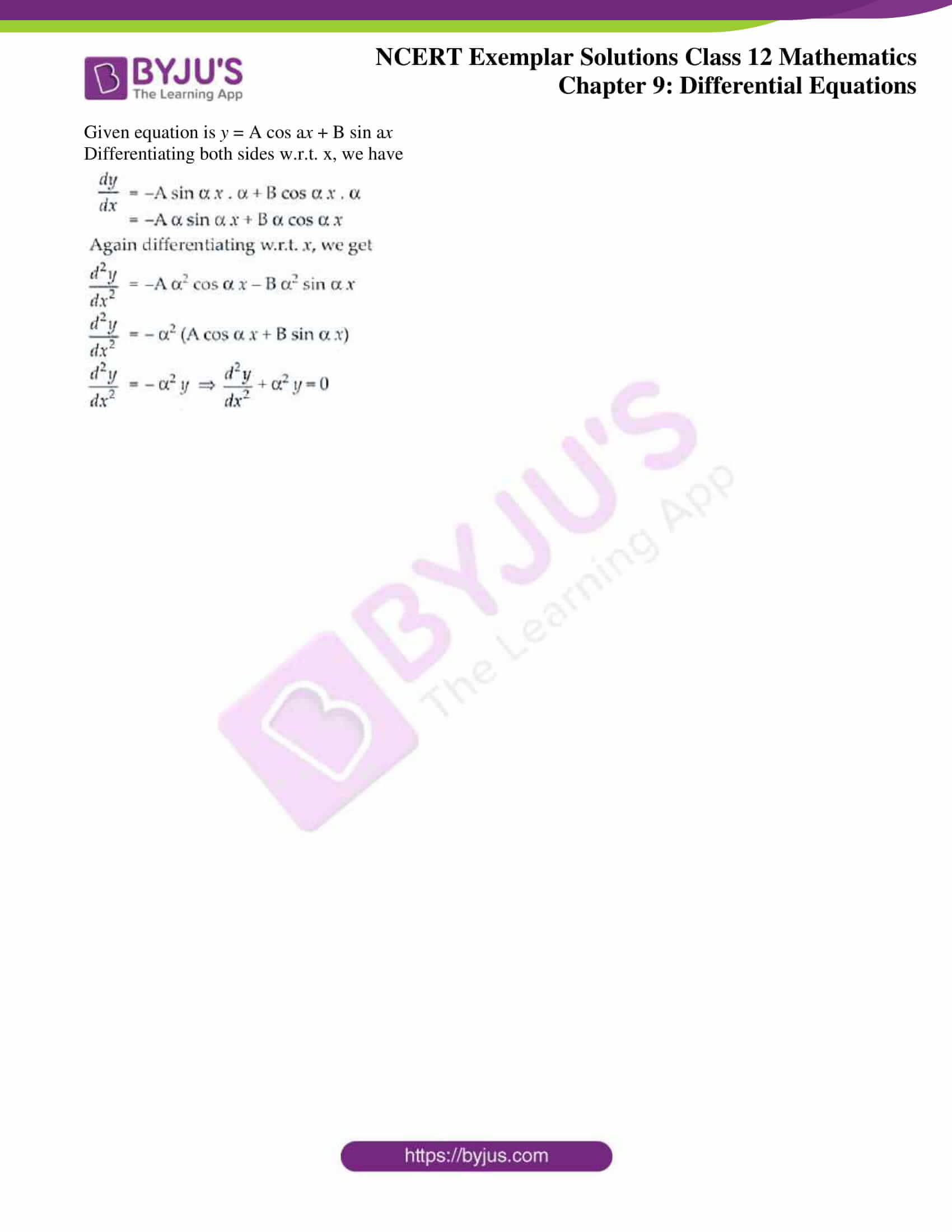### Access Answers to NCERT Exemplar Solutions for Class 12 Maths Chapter 9 Differential Equations

Exercise 9.3 Page No: 193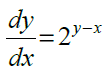1. Find the solution of

Solution: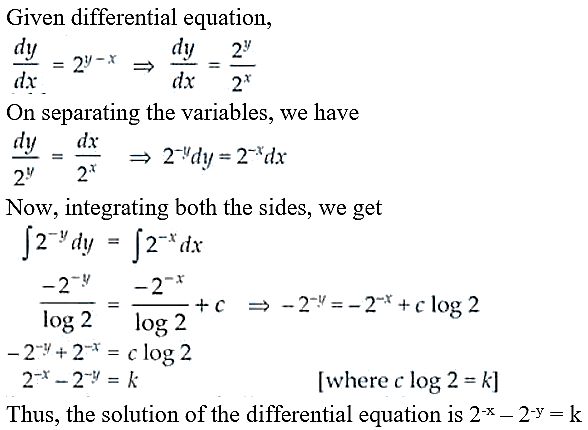2. Find the differential equation of all non-vertical lines in a plane.

Solution:

We know that, the equation of all non-vertical lines are y = mx + c

On differentiating w.r.t. x, we get

dy/dx = m

Again, on differentiating w.r.t. x, we have

d2y/dx2 = 0

Thus, the required equation is d2y/dx2 = 0.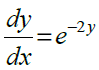3. Given that and y = 0 when x = 5.

Find the value of x when y = 3.

Solution: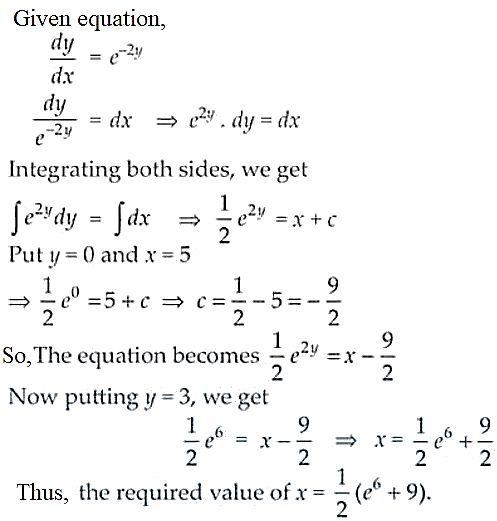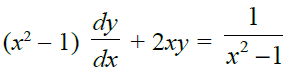4. Solve the differential equation

Solution: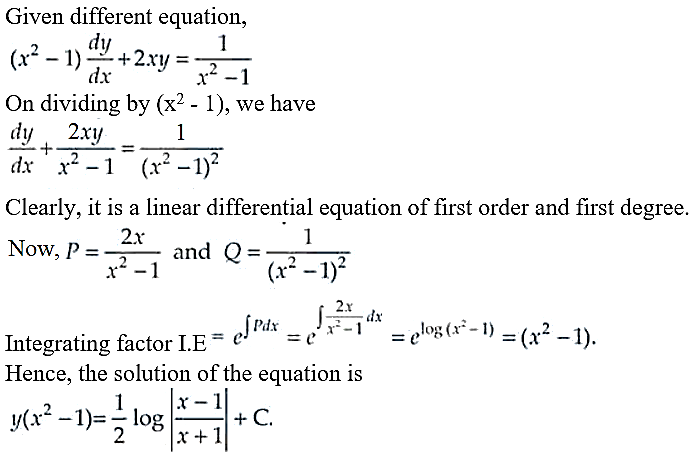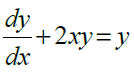5. Solve the differential equation

Solution:

Given differential equation,

dy/dx + 2xy = y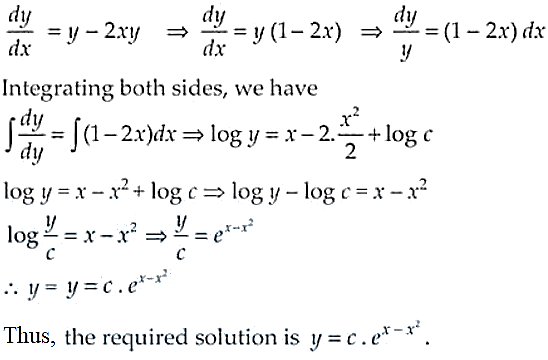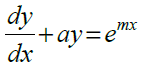6. Find the general solution of

Solution:

Given equation, dy/dx + ay = emx

Solving for linear differential equation of first order, we have

P = a and Q = emx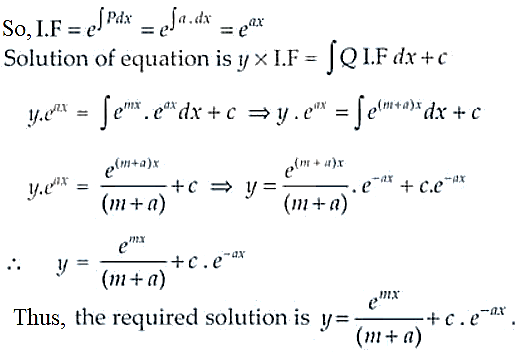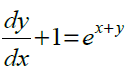7. Solve the differential equation

Solution:

Given differential equation, dy/dx + 1 = ex+y

Substituting x + y = t and differentiating w.r.t. x, we have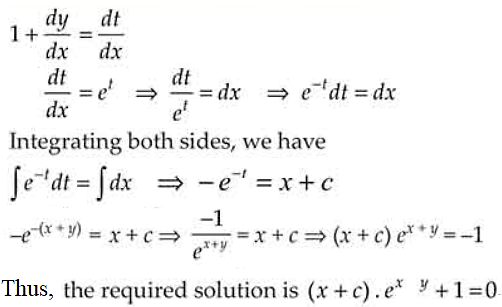8. Solve: ydx xdy = x2ydx.

Solution:

Given equation, ydx – xdy = x2ydx

y dx – x2y dx = xdy

y (1 – x2) dx = xdy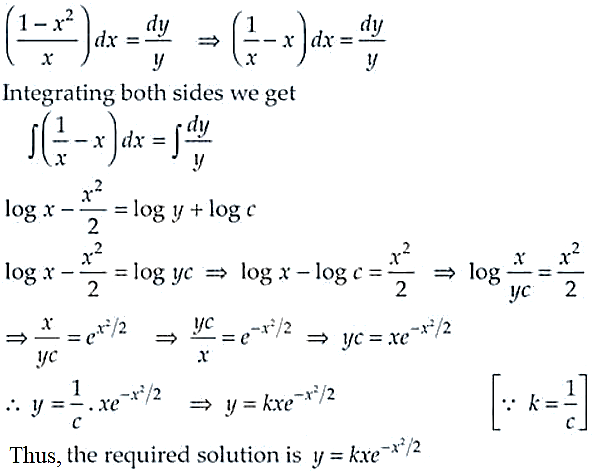9. Solve the differential equation dy/dx = 1 + x + y2 + xy2, when y = 0, x = 0.

Solution:

Given equation, dy/dx = 1 + x + y2 + xy2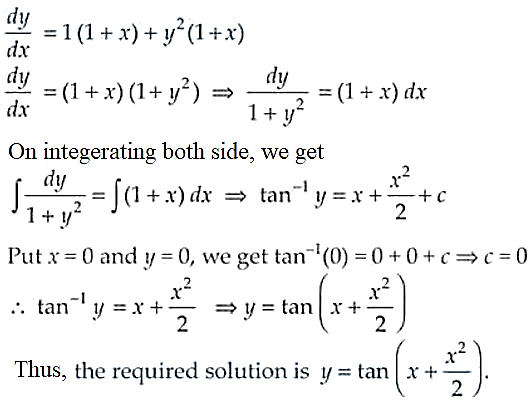10. Find the general solution of (x + 2y3) dy/dx = y.

Solution: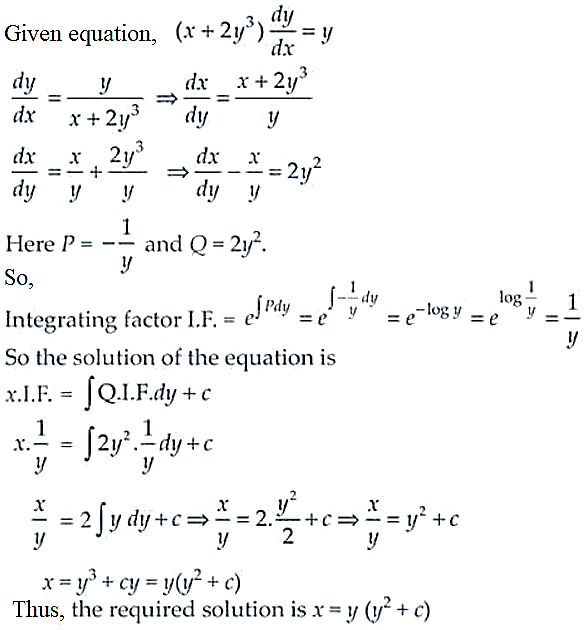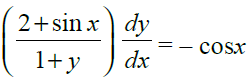11. If y(x) is a solution of and y (0) = 1, then find the value of y(π/2).

Solution: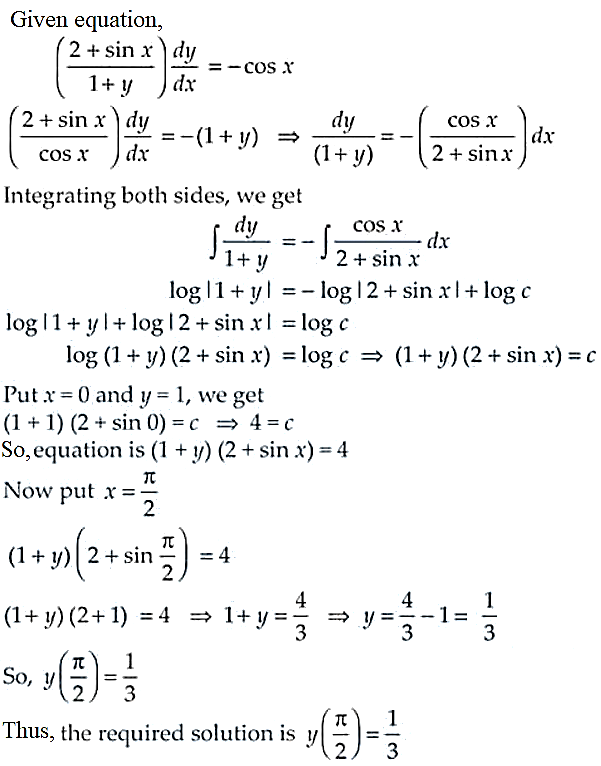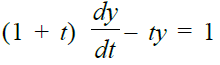12. If y(t) is a solution of and y (0) = – 1, then show that y (1) = -1/2.

Solution: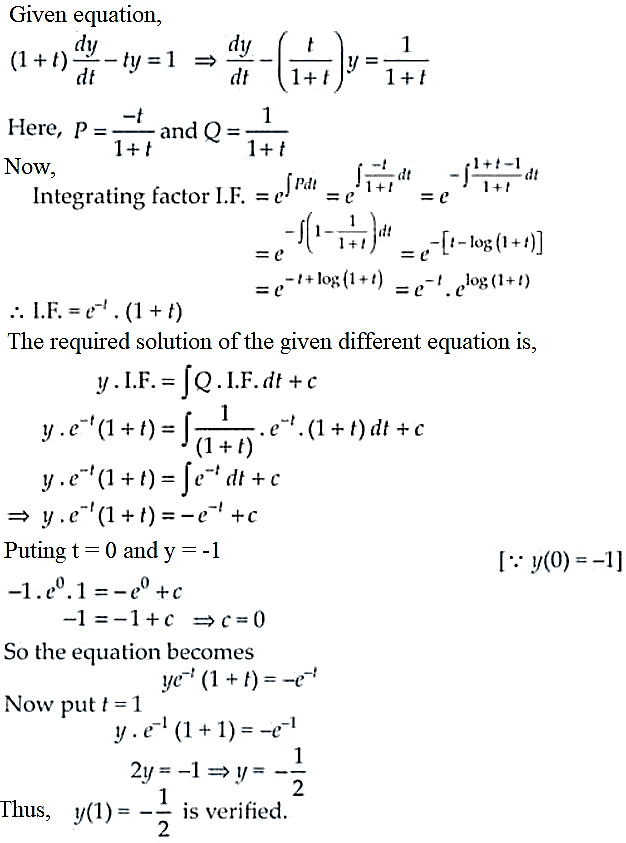13. Form the differential equation having y = (sin–1x)2 + A cos–1x + B, where A and B are arbitrary constants, as its general solution.

Solution: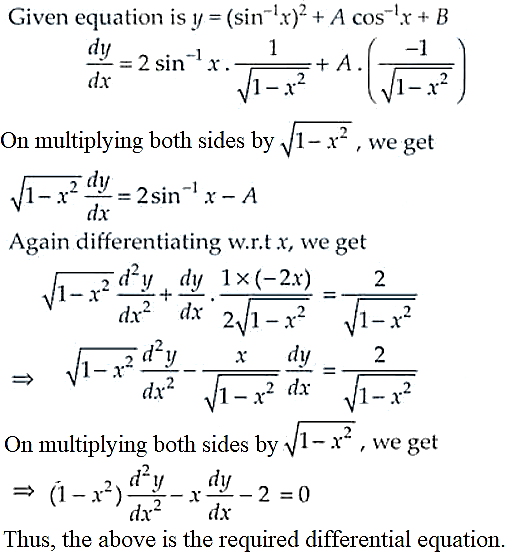14. Form the differential equation of all circles which pass through origin and whose centres lie on y-axis.

Solution:

The equation of circles which pass through the origin and whose centre lies on the y-axis is given by,

(x – 0)2 + (y – a)2 = a2, where (0, a) is the centre

x2 + y2 + a2 – 2ay = a2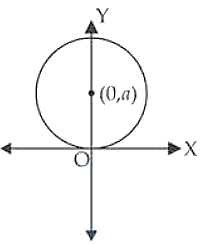x2 + y2 – 2ay = 0 ……… (i)

Differentiating both sides w.r.t. x, we get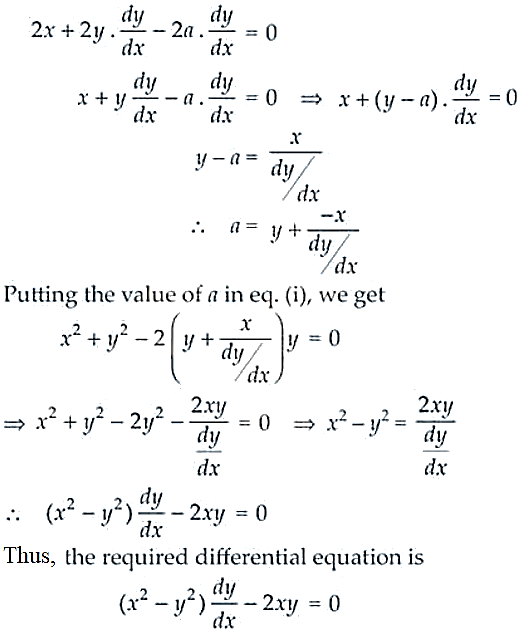15. Find the equation of a curve passing through origin and satisfying the differential equation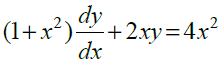Solution:

Given equation,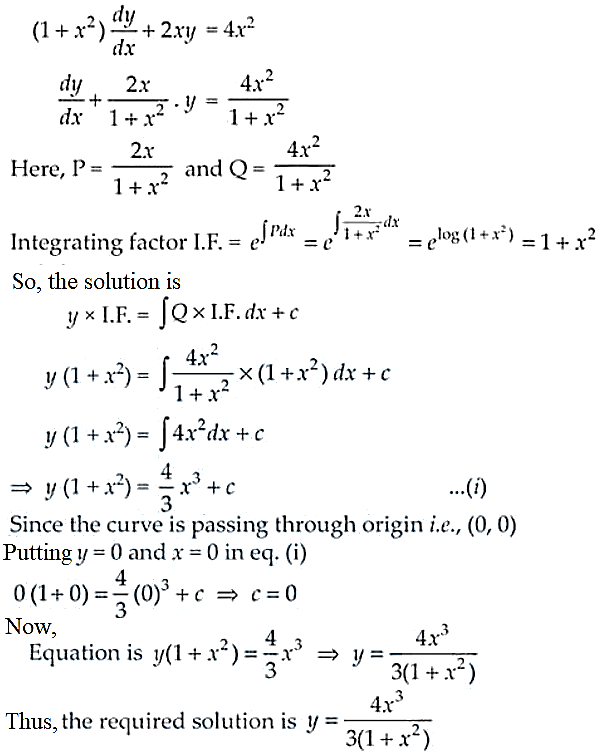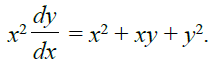16. Solve:

Solution:

Given equation,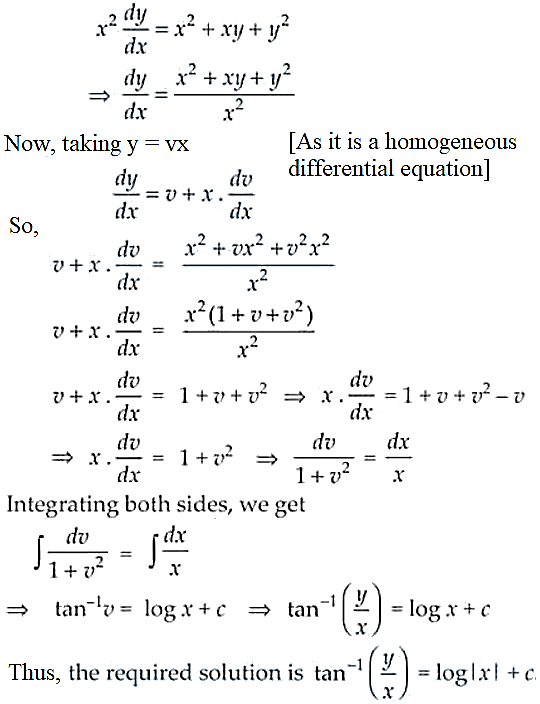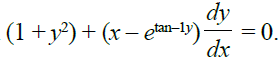17. Find the general solution of the differential equation

Solution:

Given differential equation,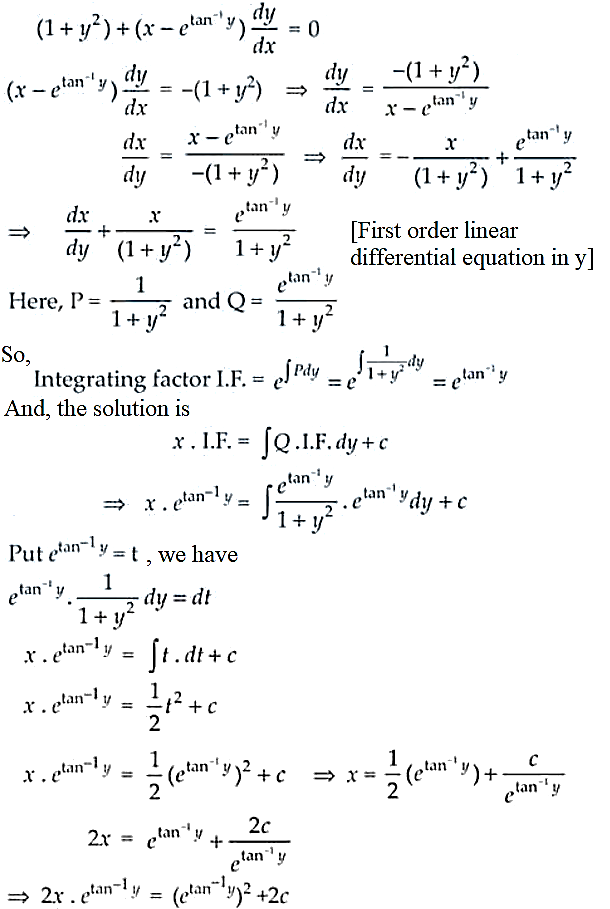Thus, the above is the required solution of the given differential equation.

18. Find the general solution of y2dx + (x2xy + y2) dy = 0.

Solution:

Given equation, y2dx + (x2xy + y2) dy = 0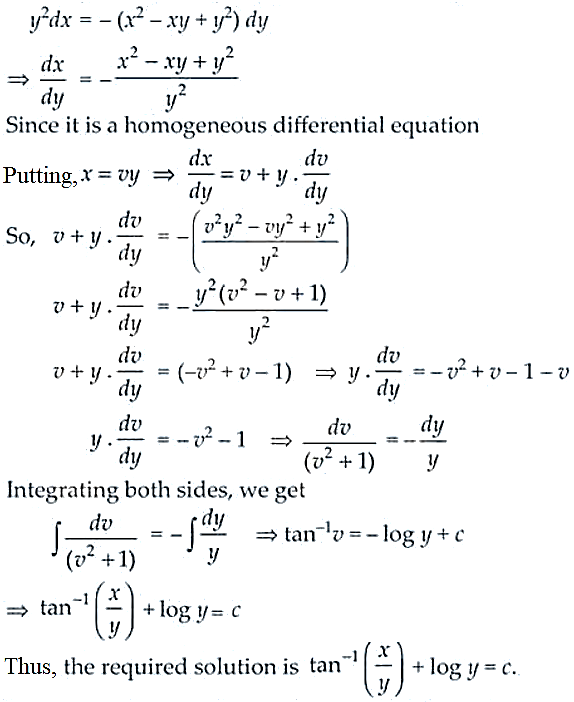19. Solve : (x + y) (dx dy) = dx + dy. [Hint: Substitute x + y = z after separating dx and dy]

Solution:

Given differential equation, (x + y) (dx dy) = dx + dy

(x + y) dx – (x – y) dy = dx + dy

– (x + y) dy – dy = dx – (x + y) dx

– (x + y + 1) dy = – (x + y – 1) dx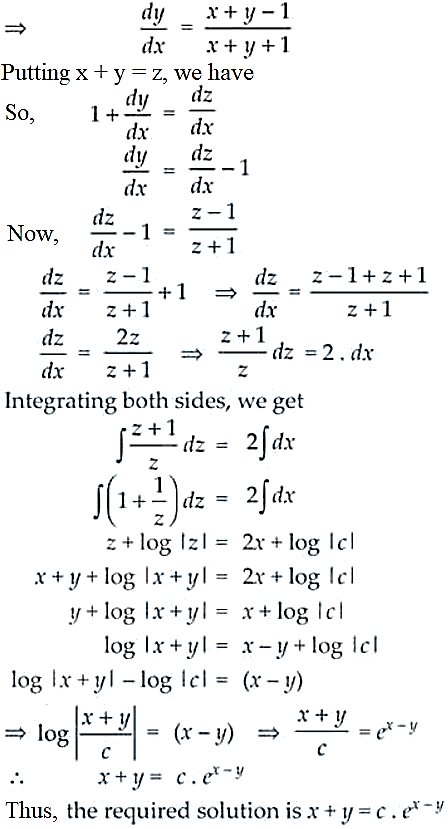20. Solve : 2 (y + 3) – xy dy/dx = 0, given that y(1) = -2.

Solution:

Given differential equation, 2 (y + 3) – xy dy/dx = 0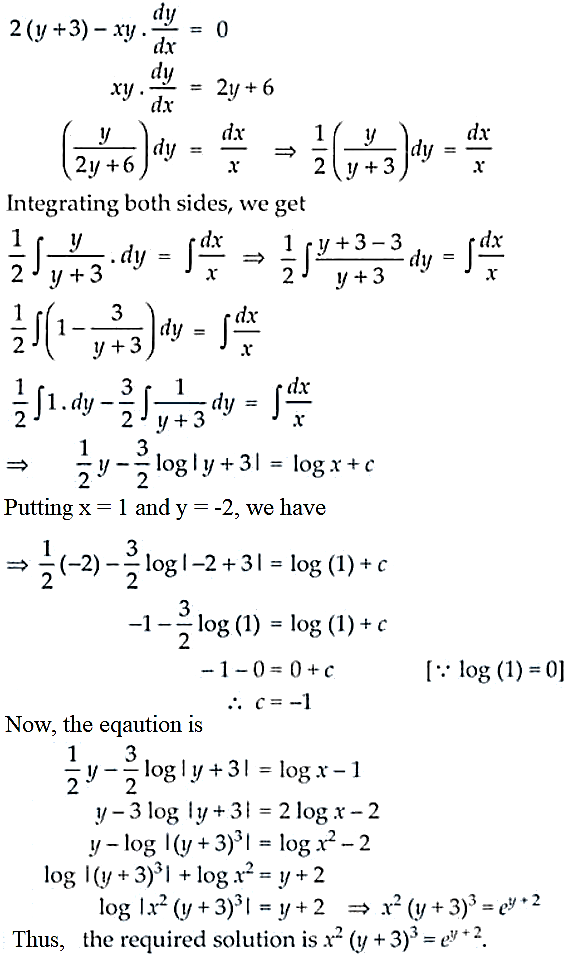21. Solve the differential equation dy = cos x (2 – y cosec x) dx given that y = 2 when x = π/2.

Solution:

Given differential equation, dy = cos x (2 – y cosec x) dx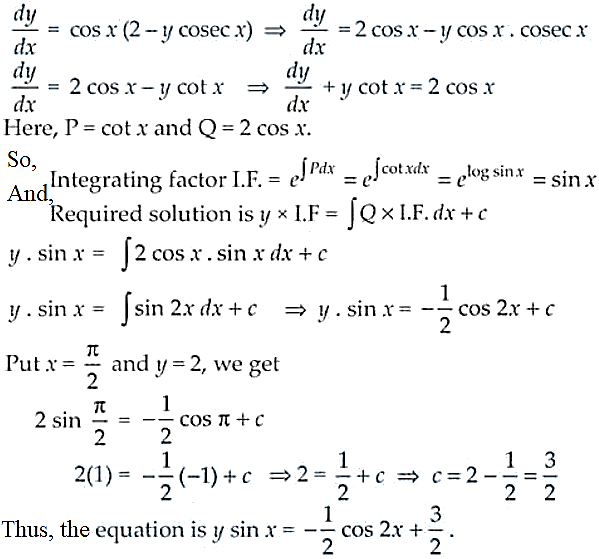22. Form the differential equation by eliminating A and B in Ax2 + By2 = 1.

Solution:

Given, Ax2 + By2 = 1

Differentiating w.r.t. x, we get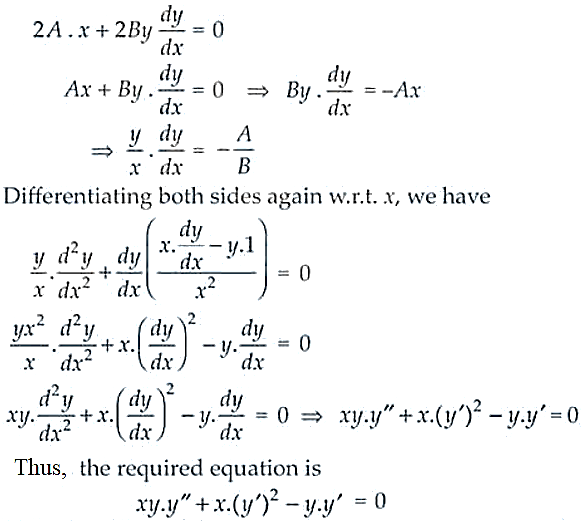23. Solve the differential equation (1 + y2) tan–1x dx + 2y (1 + x2) dy = 0.

Solution:

Given differential equation, (1 + y2) tan–1x dx + 2y (1 + x2) dy = 0

2y (1 + x2) dy = -(1 + y2) tan-1x dx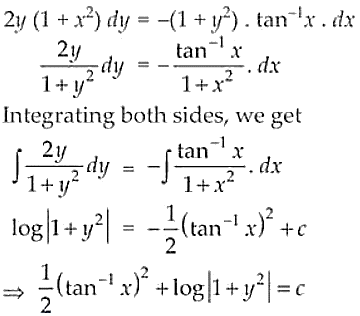Thus, the above equation is the required solution.

24. Find the differential equation of system of concentric circles with centre (1, 2).

Solution:

The family of concentric circles with centre (1, 2) and radius ‘r’ is given by

(x – 1)2 + (y – 2)2 = r2

Differentiating both sides w.r.t, x we get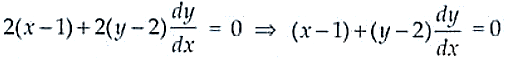Thus, the above is the required equation.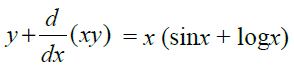25. Solve :

Solution:

Given differential equation,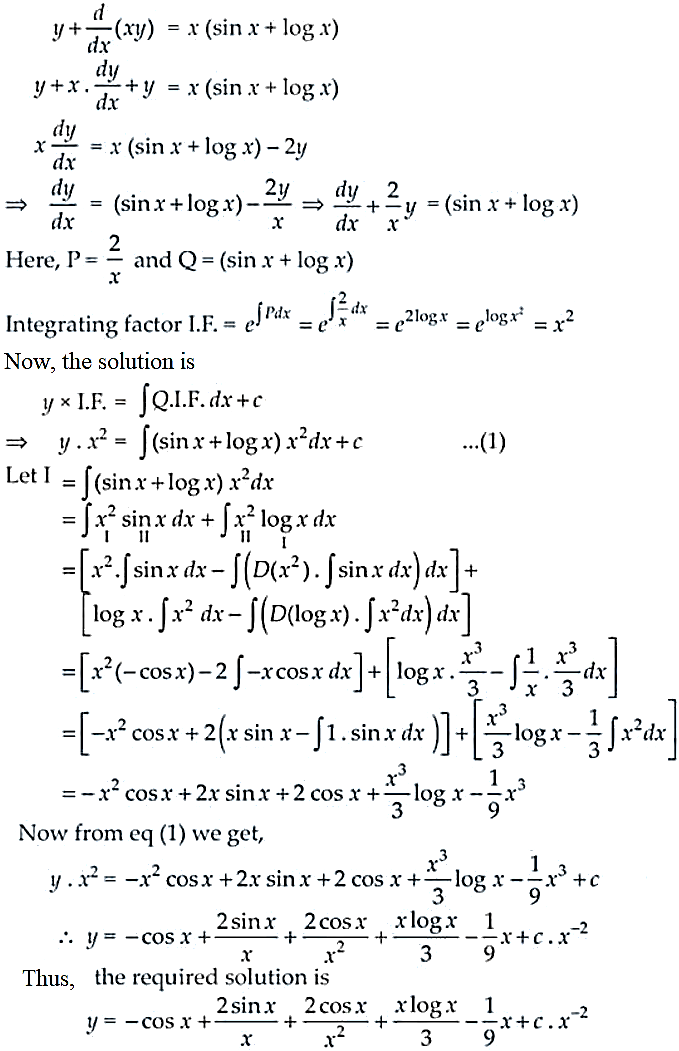26. Find the general solution of (1 + tan y) (dx dy) + 2xdy = 0.

Solution:

Given, (1 + tan y) (dx dy) + 2xdy = 0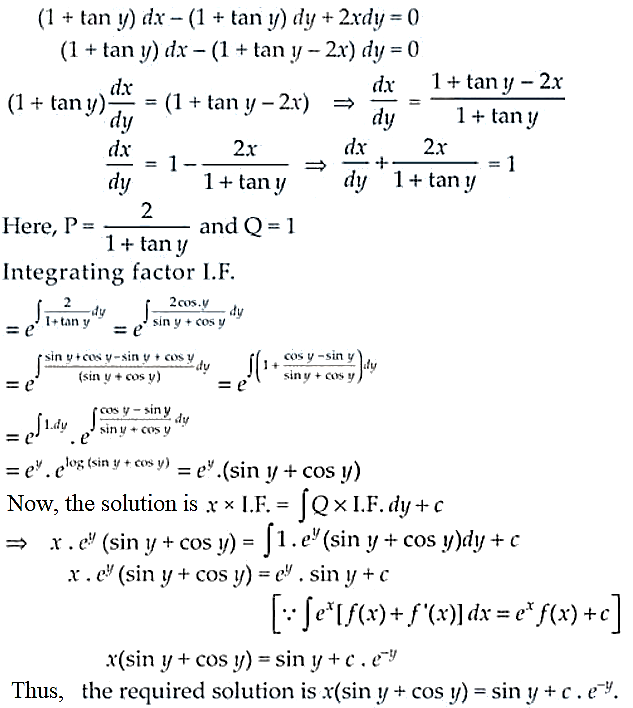27. Solve: dy/dx = cos(x + y) + sin (x + y). [Hint: Substitute x + y = z]

Solution: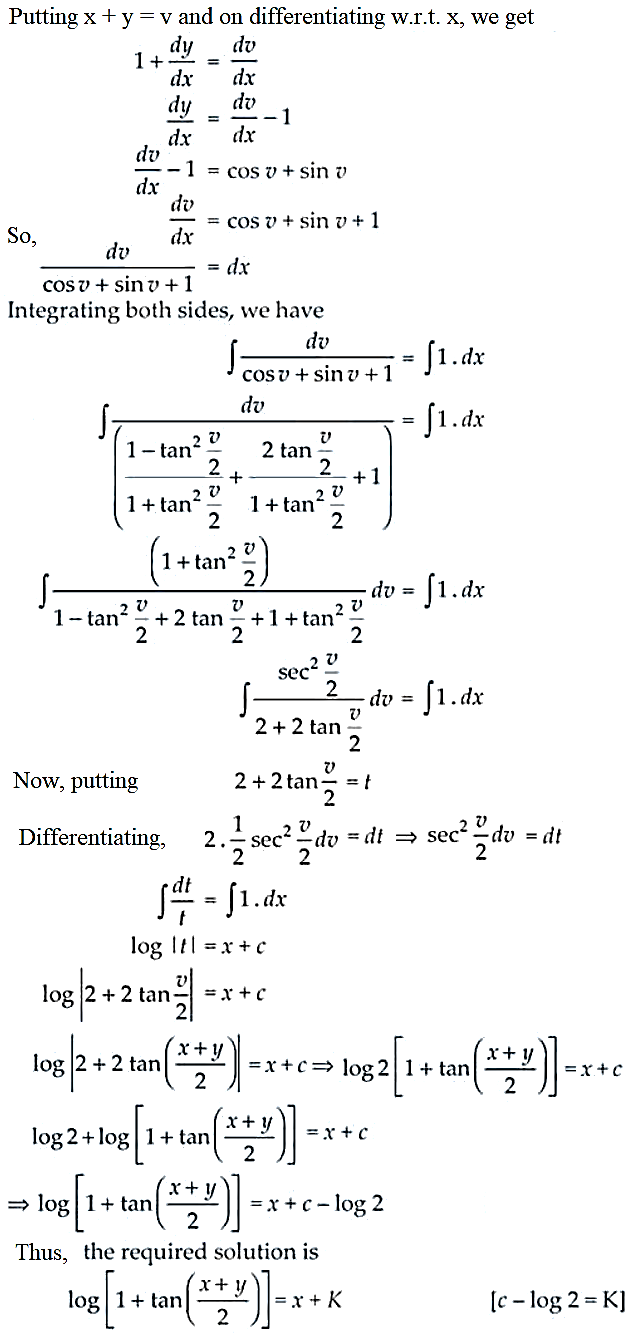28. Find the general solution of dy/dx – 3y = sin 2x.

Solution:

Given equation, dy/dx – 3y = sin 2x

It’s a first order linear differential equation

Here P = -3 and Q = sin 2x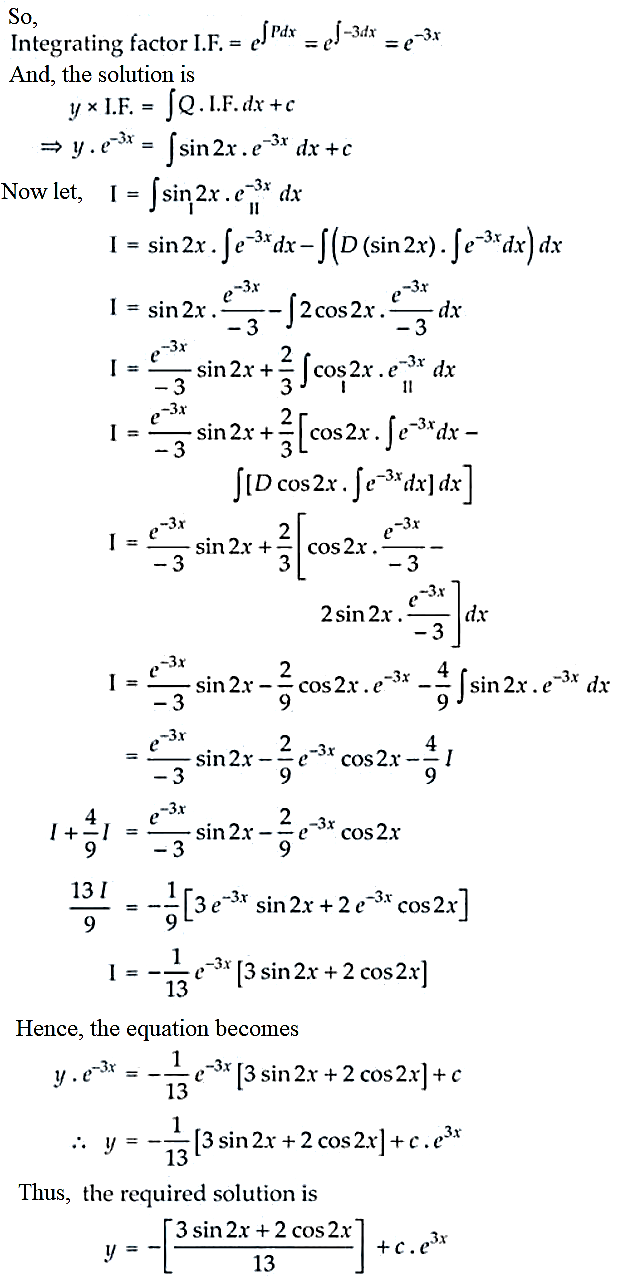29. Find the equation of a curve passing through (2, 1) if the slope of the tangent to the curve at any point (x, y) is (x2 + y2)/ 2xy.

Solution:

Given that the slope of tangent to a curve at (x, y) is (x2 + y2)/ 2xy.

It’s a homogeneous differential function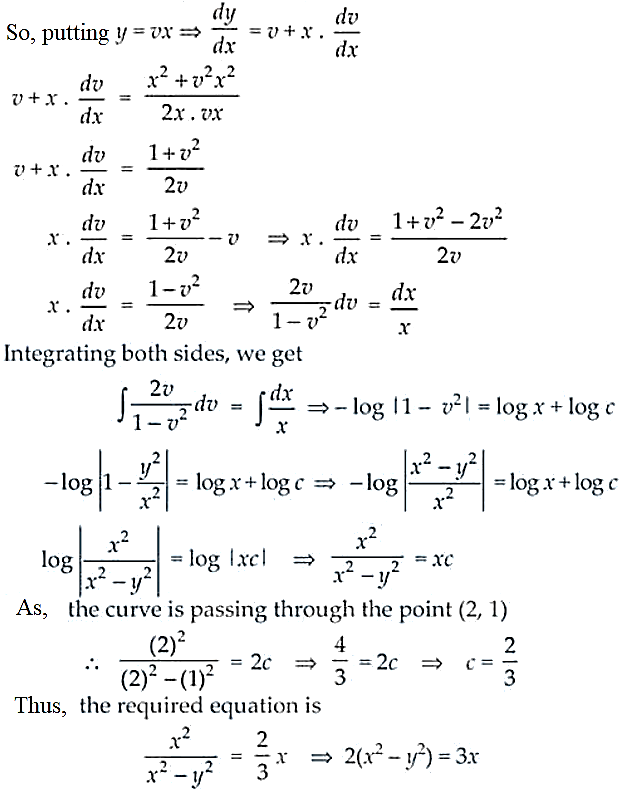30. Find the equation of the curve through the point (1, 0) if the slope of the tangent to the curve at any point (x, y) is (y – 1)/ (x2 + x)

Solution:

Given that the slope of the tangent to the curve at (x, y) is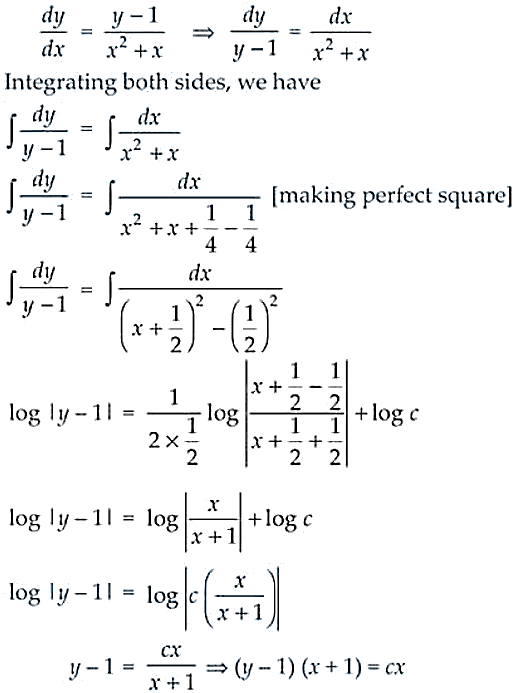As, the line is passing through the point (1, 0), then (0 – 1) (1 + 1) = c(1) ⇒ c = 2

Thus, the required solution is (y – 1) (x + 1) = 2x.

31. Find the equation of a curve passing through origin if the slope of the tangent to the curve at any point (x, y) is equal to the square of the difference of the abscissa and ordinate of the point.

Solution:

We know that,

The slope of the tangent of the curve = dy/dx

And the difference between the abscissa and ordinate = x – y

So, as given in the question we have

dy/dx = (x – y)2

Taking, x – y = v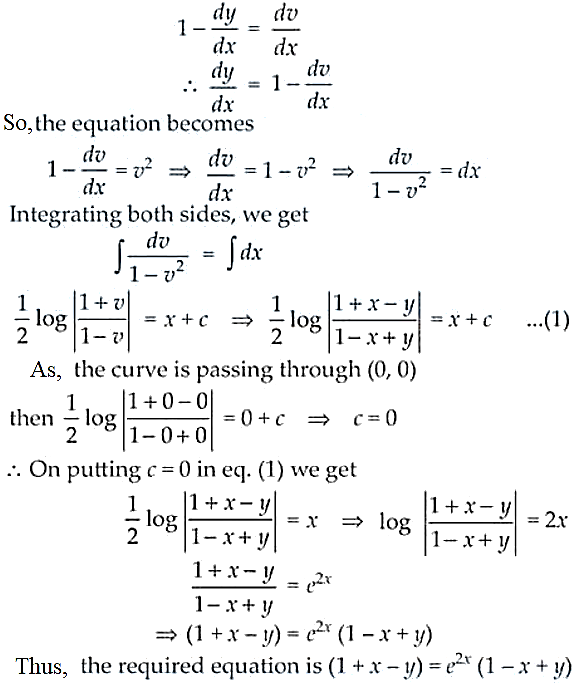32. Find the equation of a curve passing through the point (1, 1). If the tangent drawn at any point P (x, y) on the curve meets the co-ordinate axes at A and B such that P is the mid-point of AB.

Solution:

Let’s take P(x , y) be any point on the curve and AB be the tangent to the given curve at P.

Also given, P is the mid-point of AB

So, the coordinates of A and B are (2x, 0) and (0, 2y) respectively.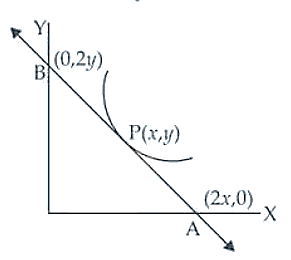The slope of the tangent AB = (2y – 0)/ (0 – 2x) = -y/x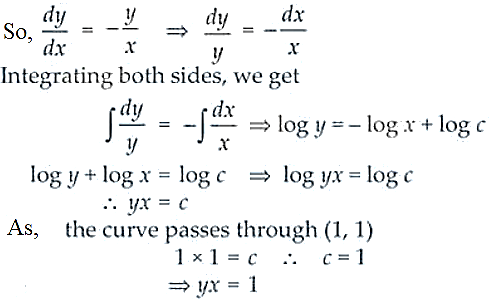Thus, the required equation is xy = 1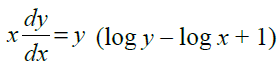33. Solve :

Solution: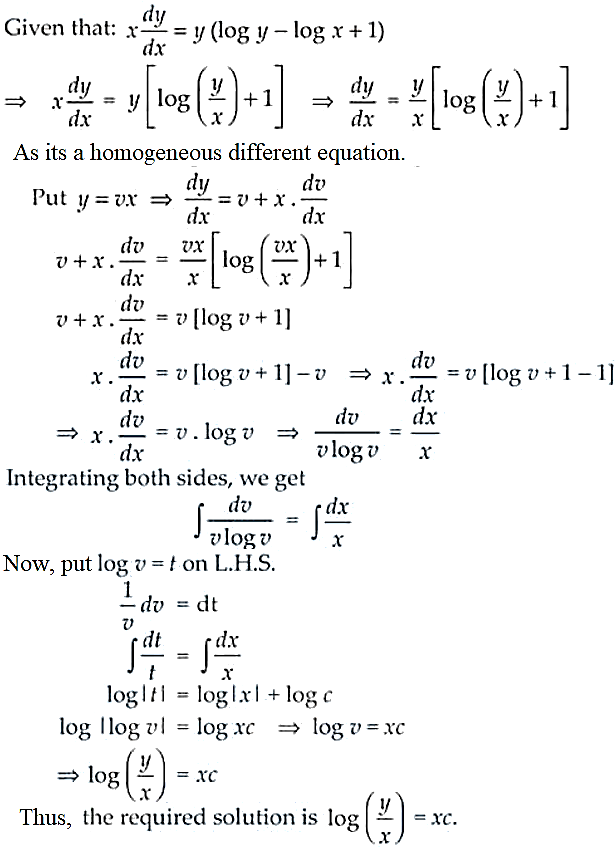Objective Type

Choose the correct answer from the given four options in each of the Exercises from 34 to 75 (M.C.Q)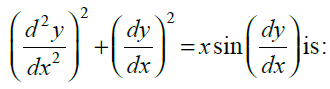34. The degree of the differential equation

(A) 1 (B) 2 (C) 3 (D) not defined

Solution:

Correct option is (D) not defined.

Since the value of sin (dy/dx) on expansion will be in increasing power of dy/dx, the degree of the given differential equation is not defined.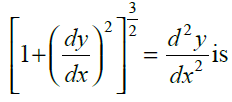35. The degree of the differential equation

(A) 4 (B) 3/2 (C) not defined (D) 2

Solution:

Correct option is (D) 2.

Given differential equation is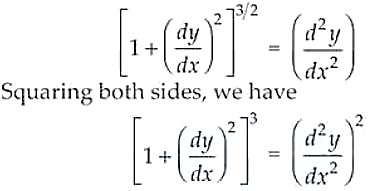Thus, the degree of the given differential equation is 2.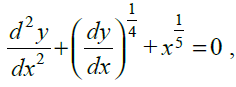36. The order and degree of the differential equation respectively, are

(A) 2 and not defined (B) 2 and 2 (C) 2 and 3 (D) 3 and 3

Solution:

Correct option is (A) 2 and not defined.

Given differential equation is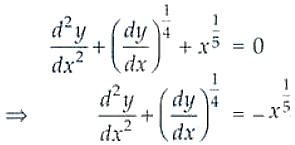As the degree of dy/dx is in fraction its undefined and the degree is 2.

37. If y = ex (A cos x + B sin x), then y is a solution of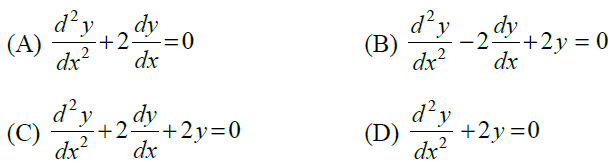Solution:

Correct option is (C).

Given equation, y = ex (A cos x + B sin x)

Differentiating on both the sides, w.r.t. x, we get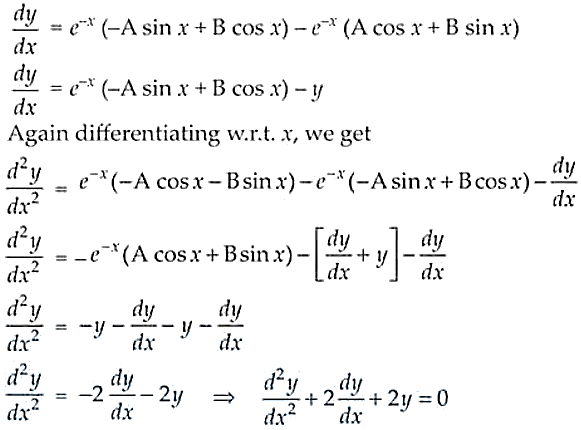38. The differential equation for y = A cos αx + B sin αx, where A and B are arbitrary constants is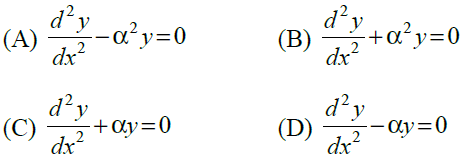Solution:

Correct option is (B).

Given equation is y = A cos ax + B sin ax

Differentiating both sides w.r.t. x, we have